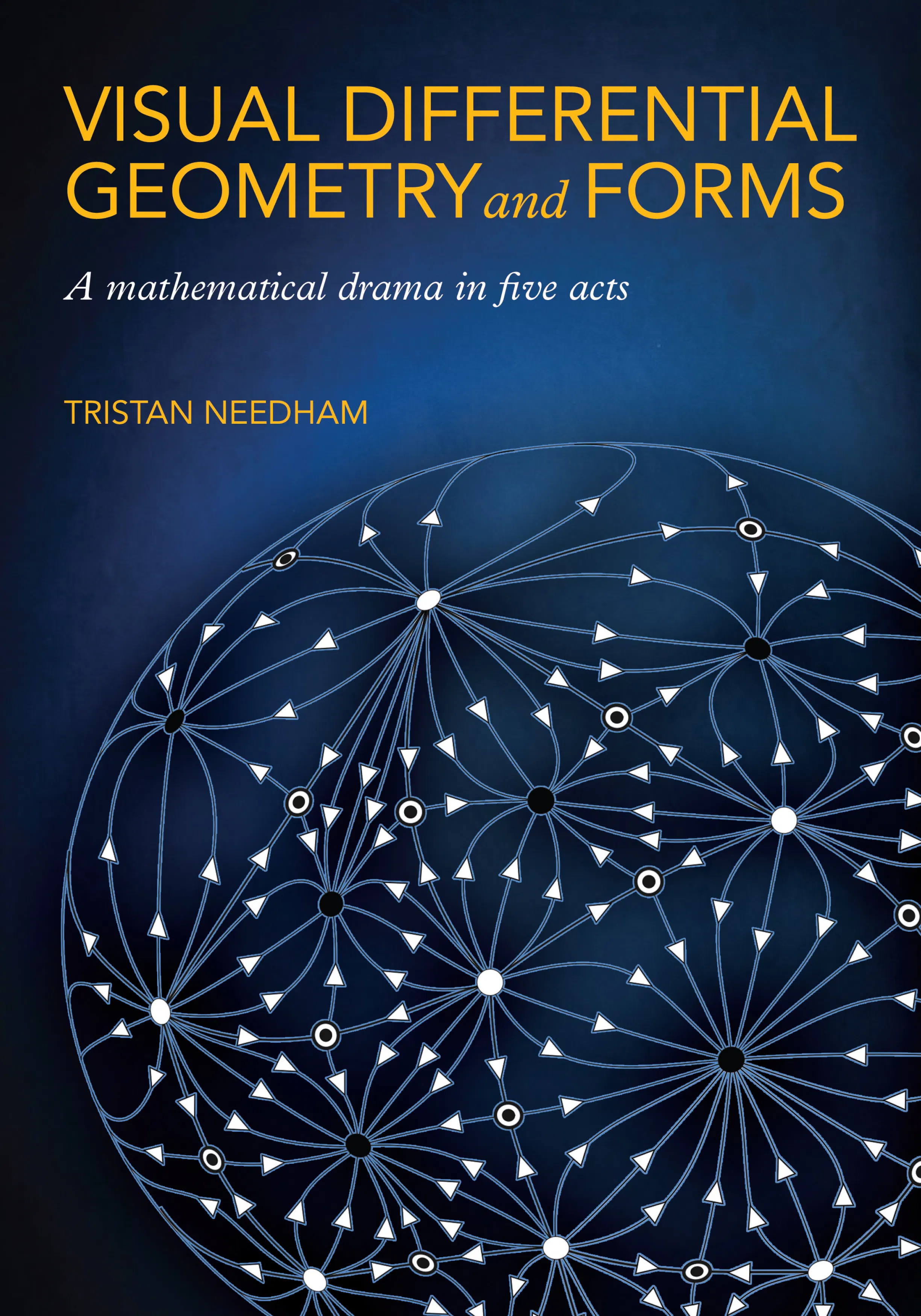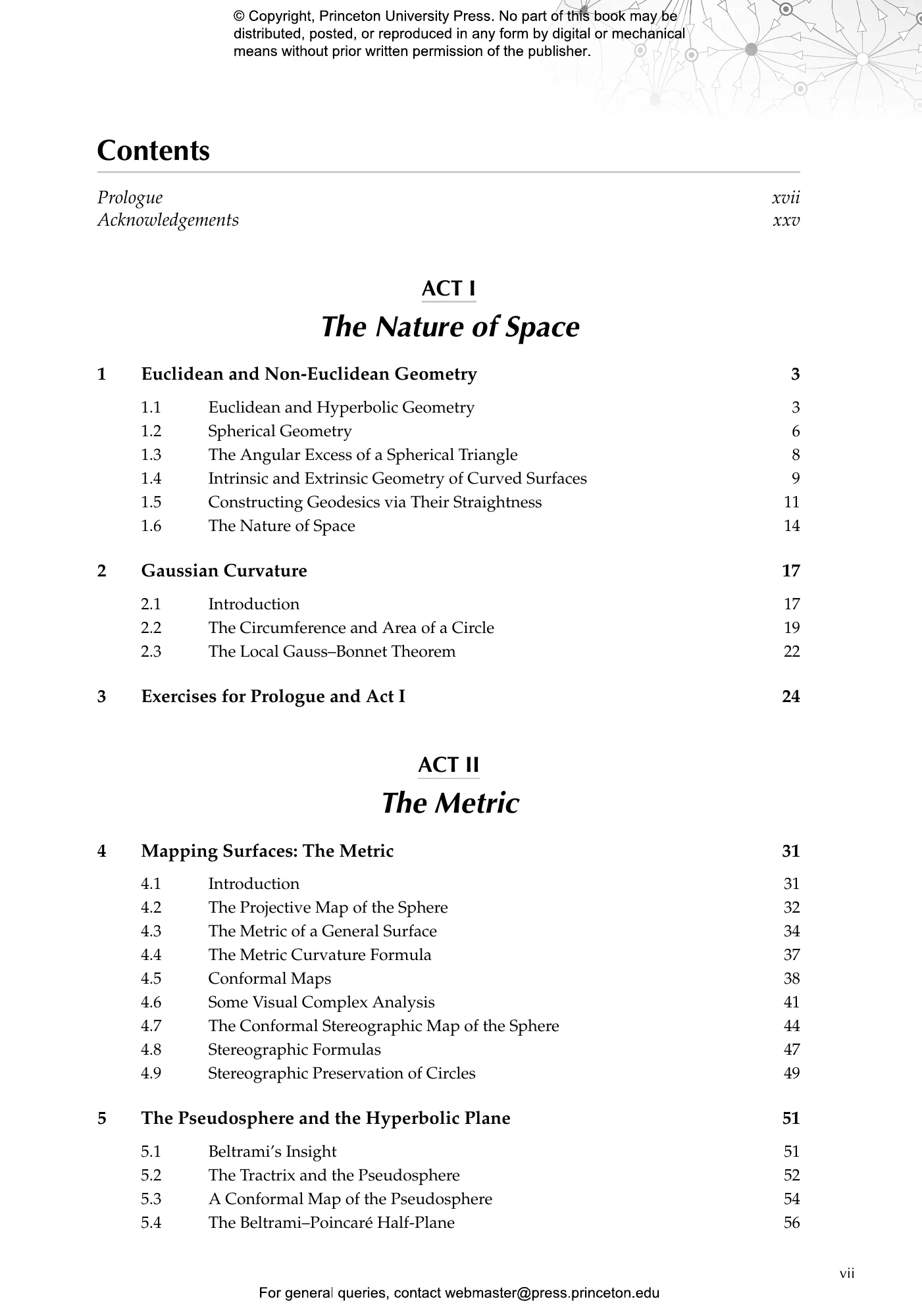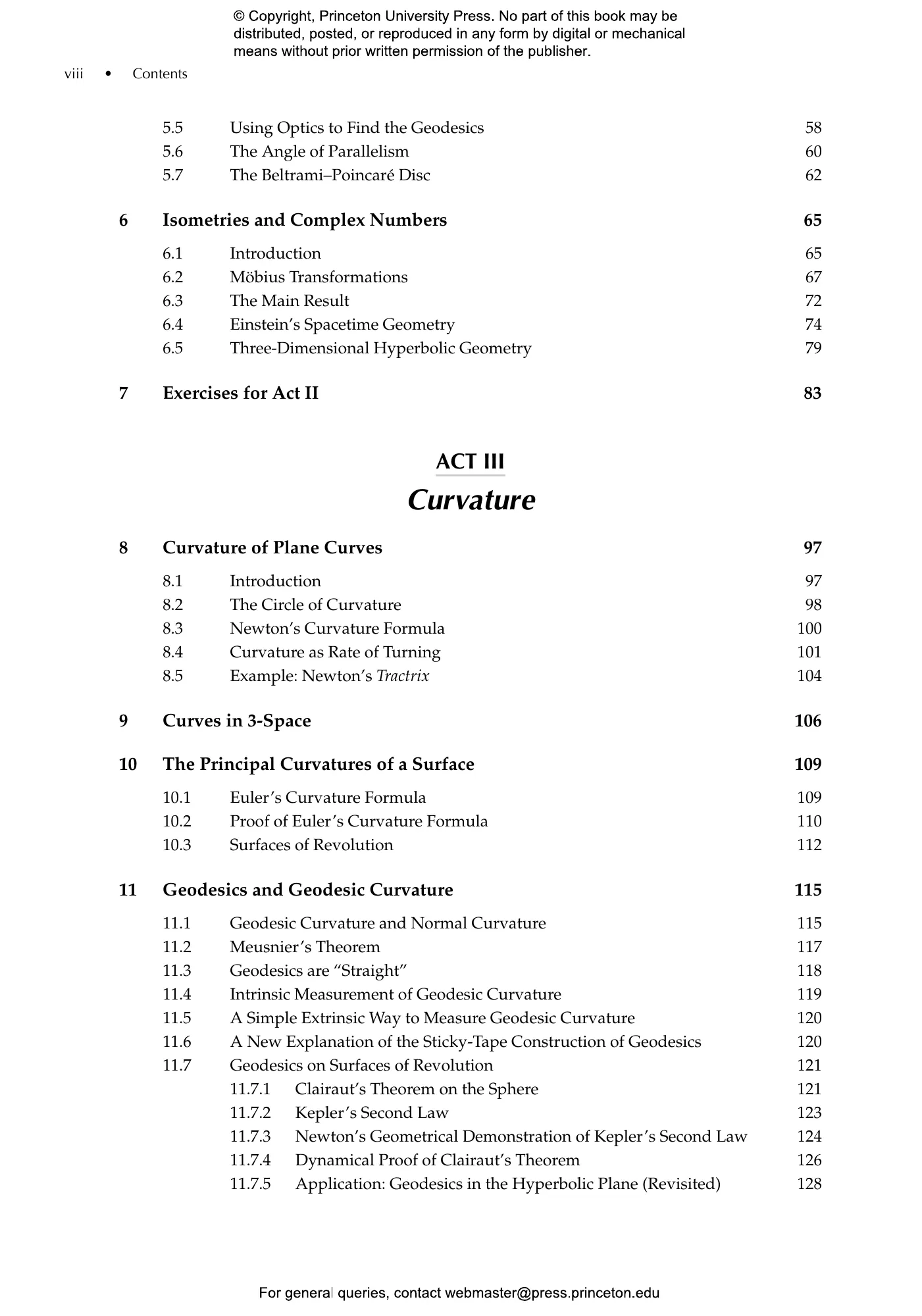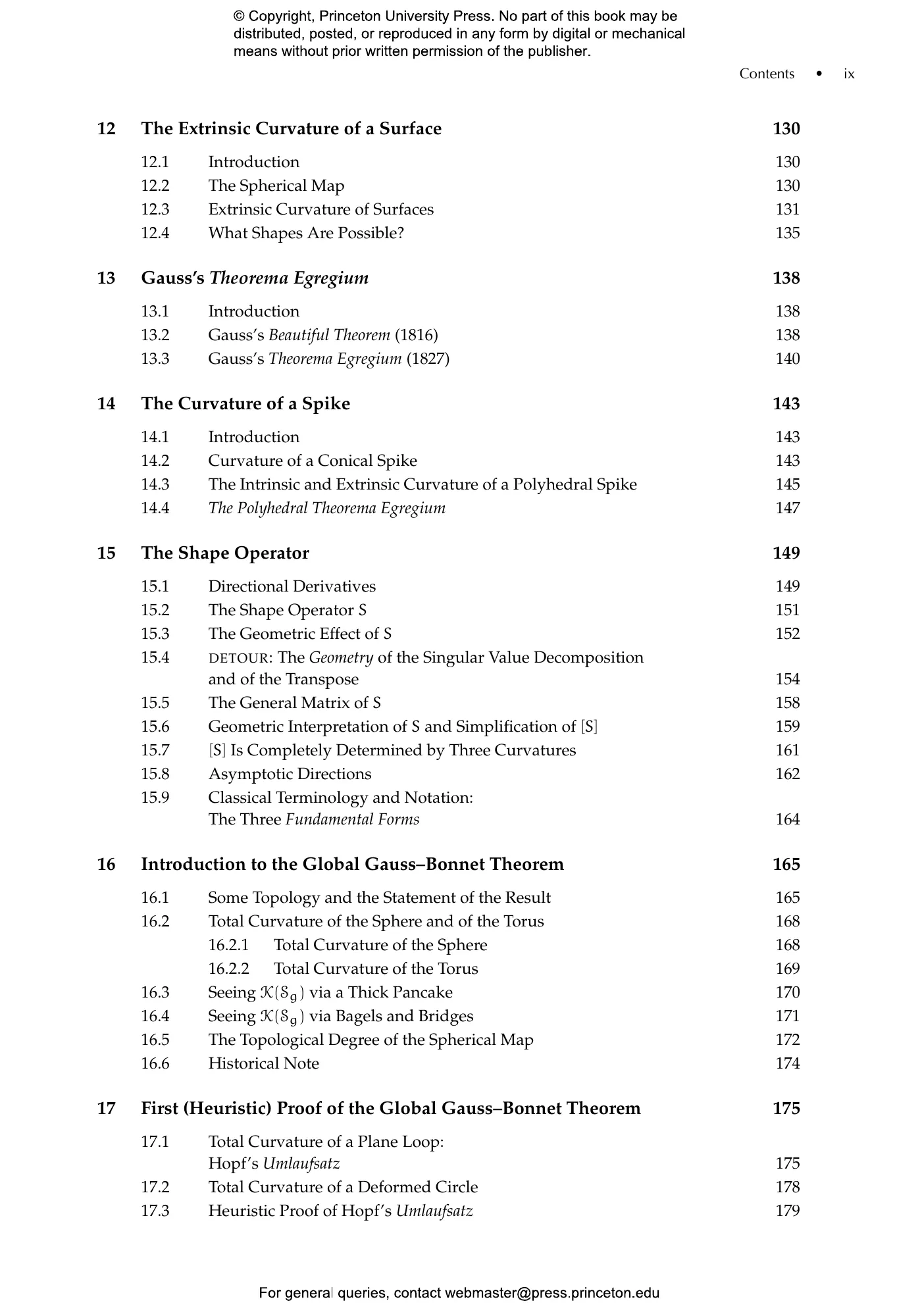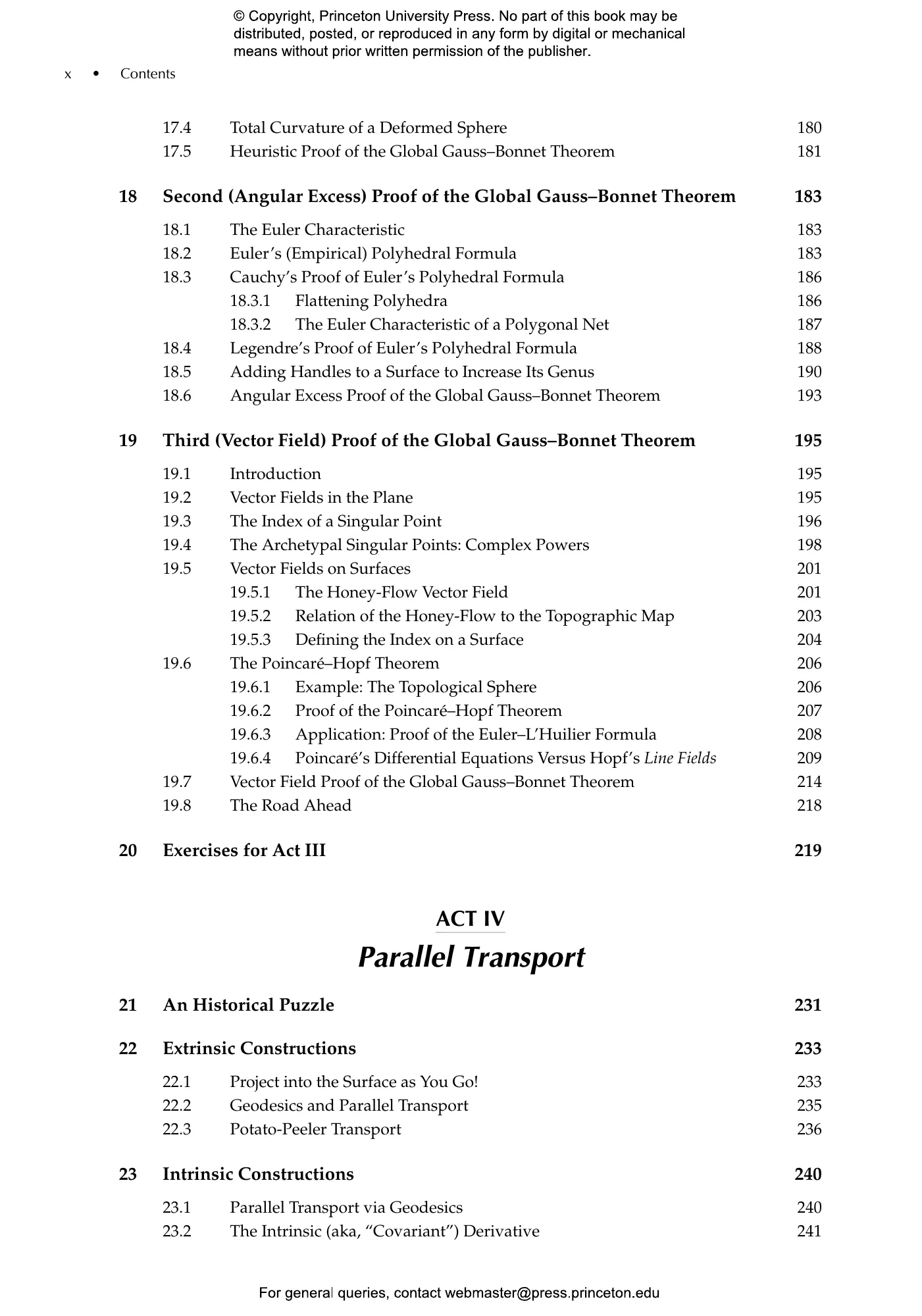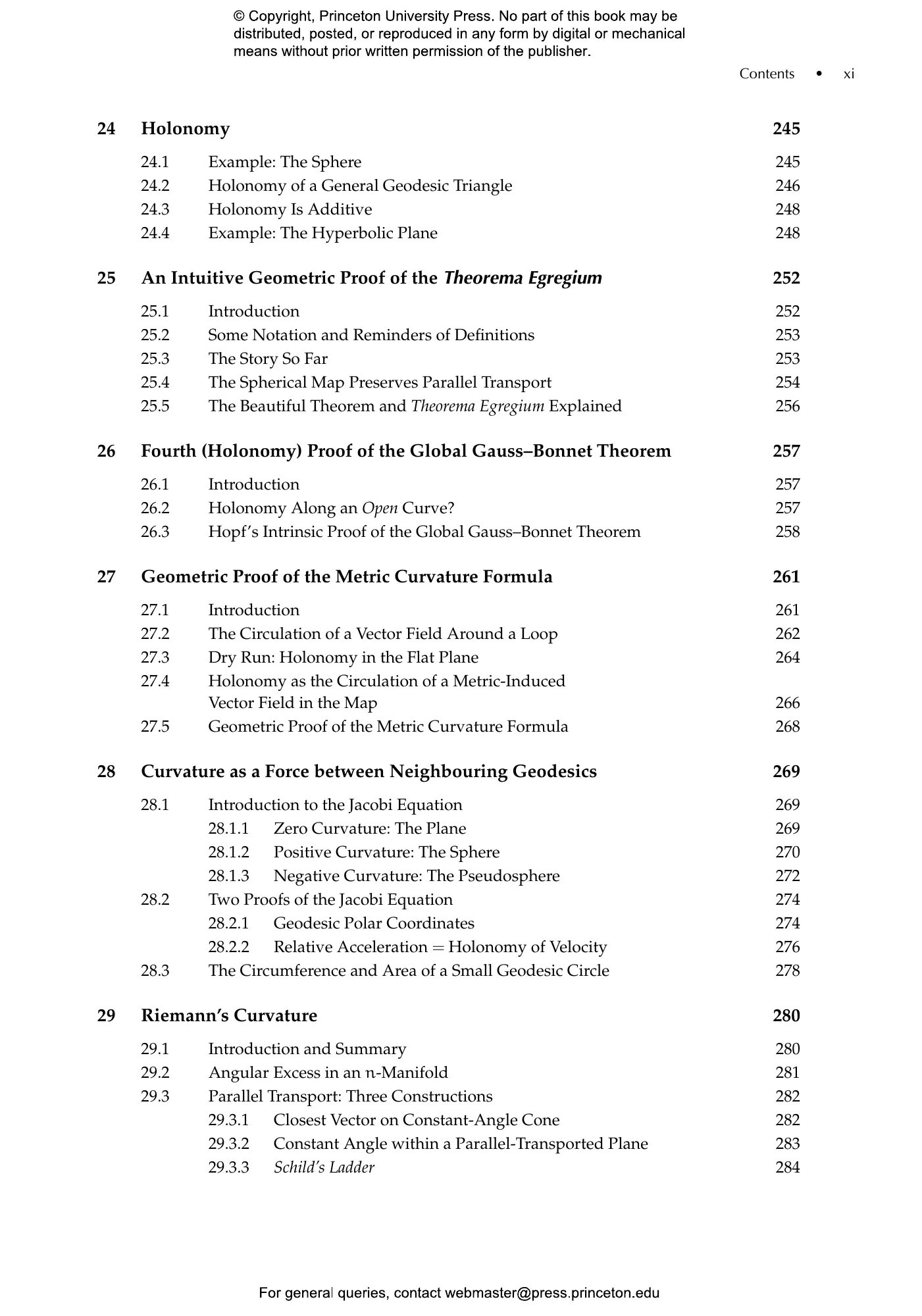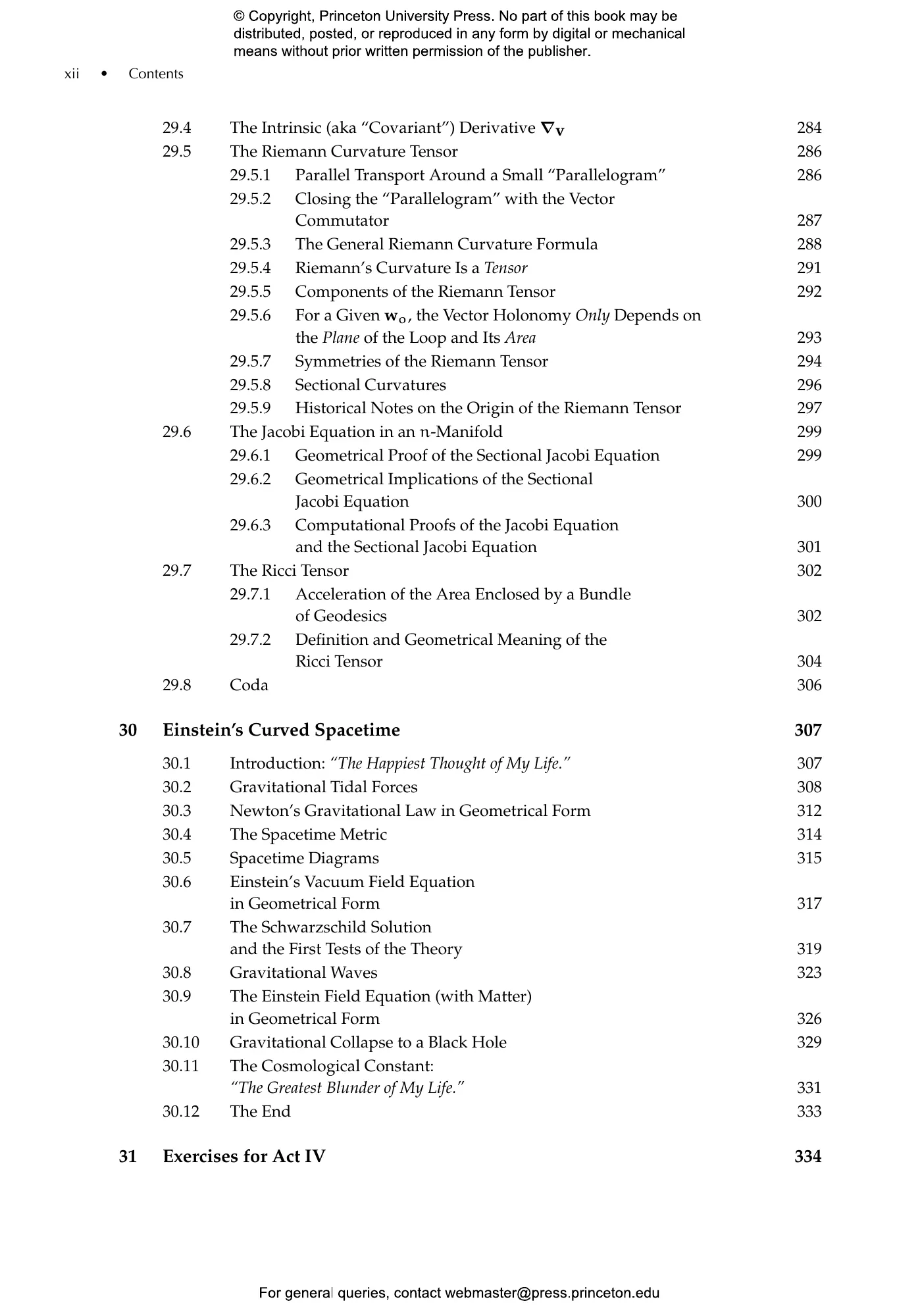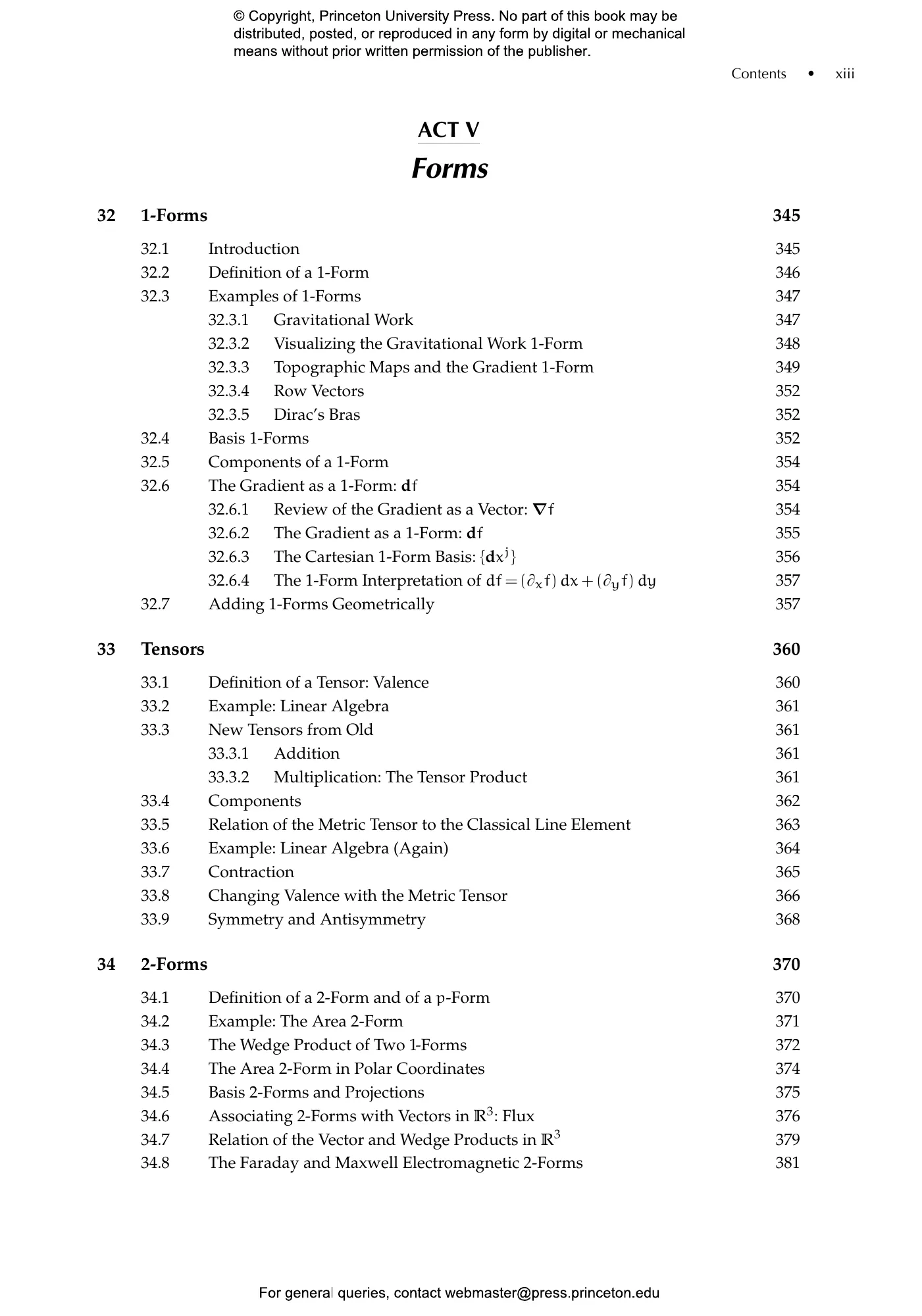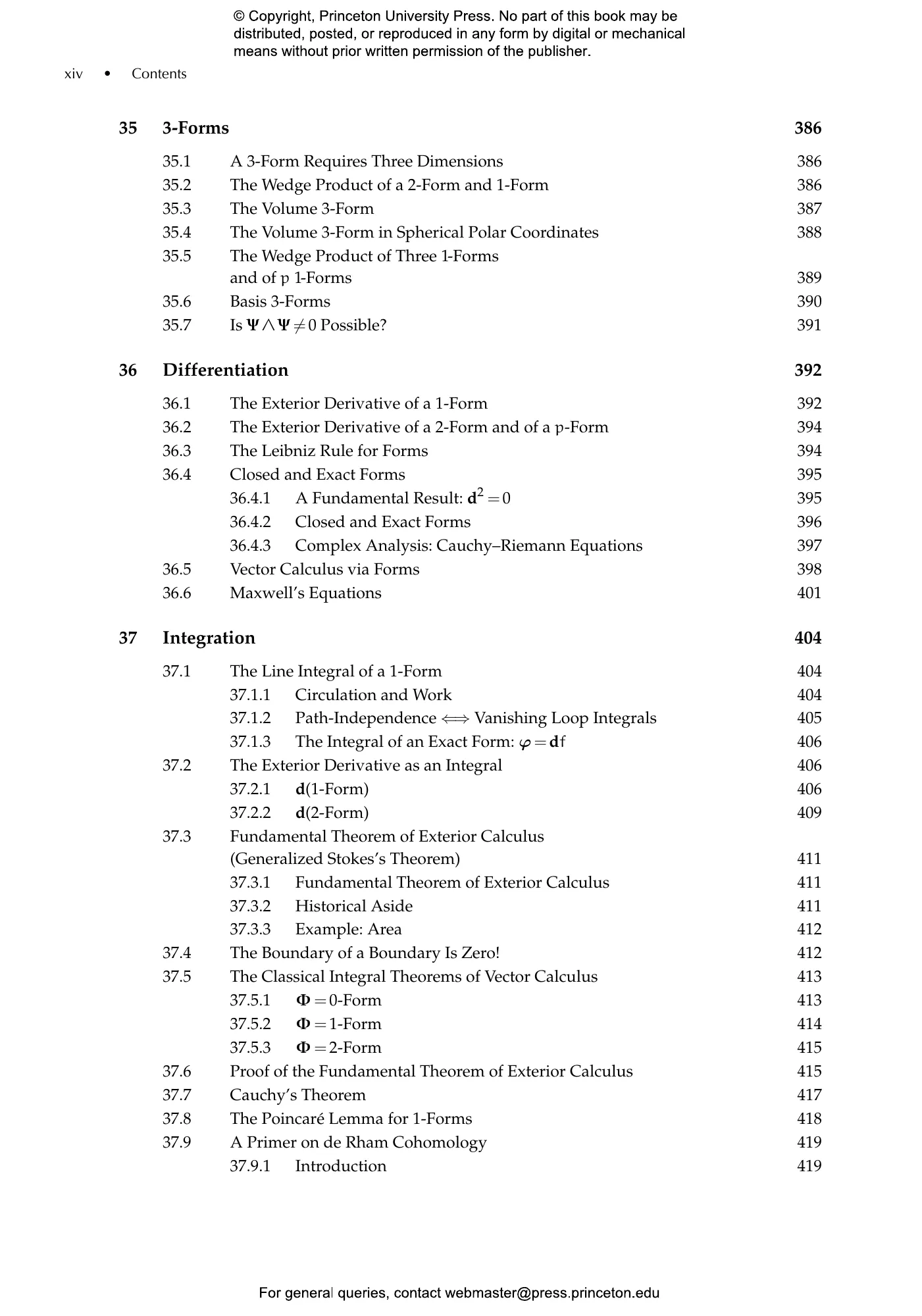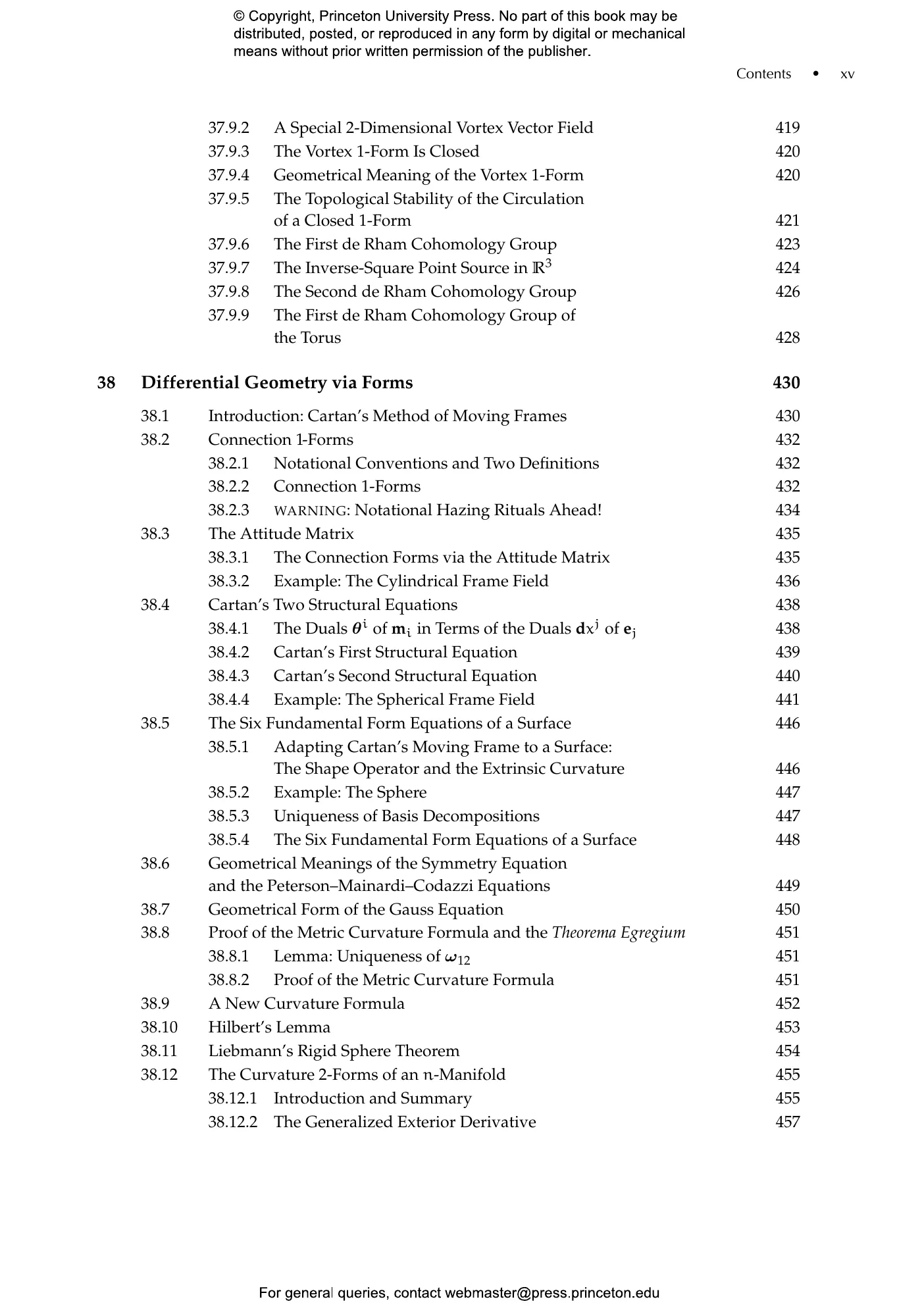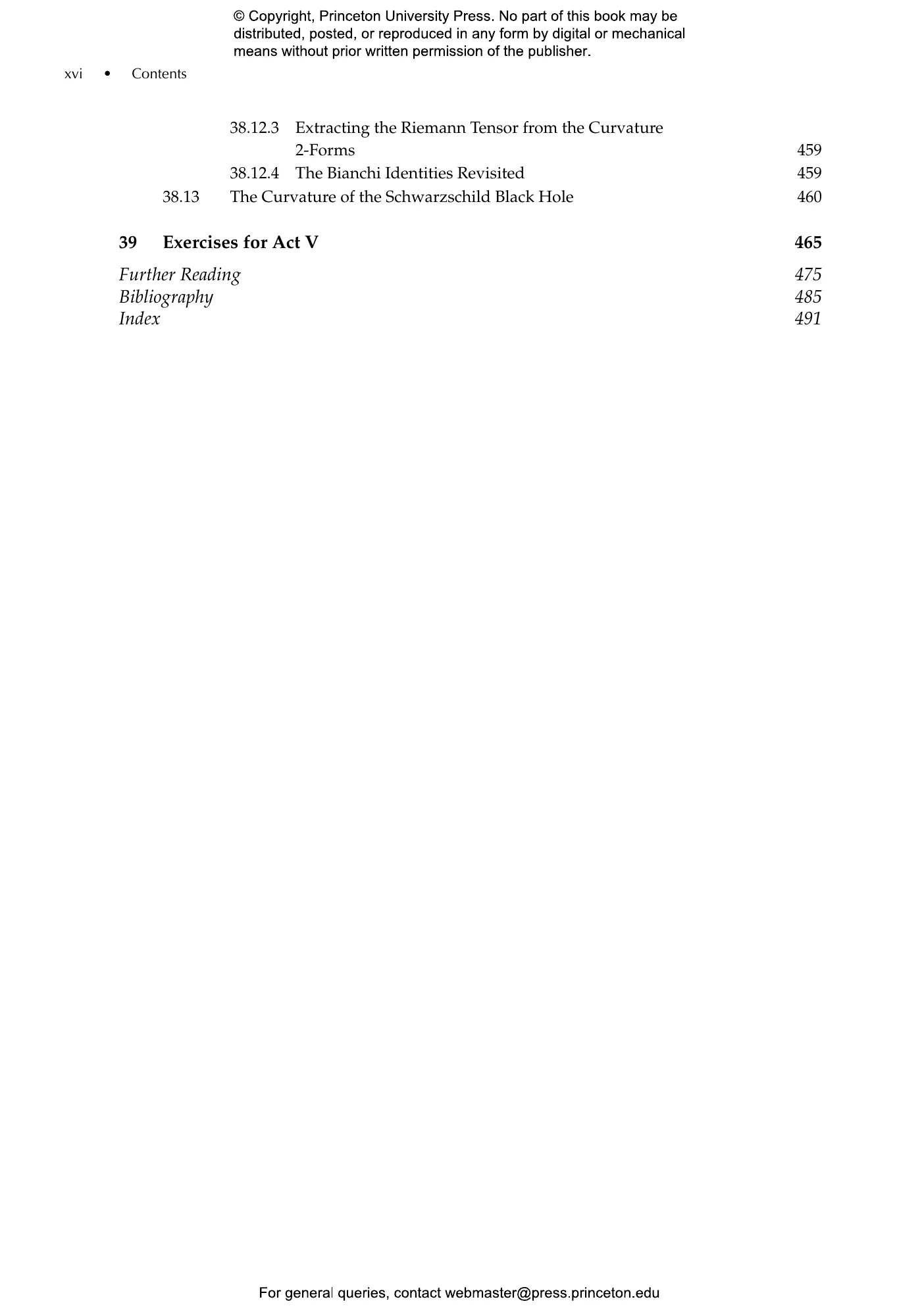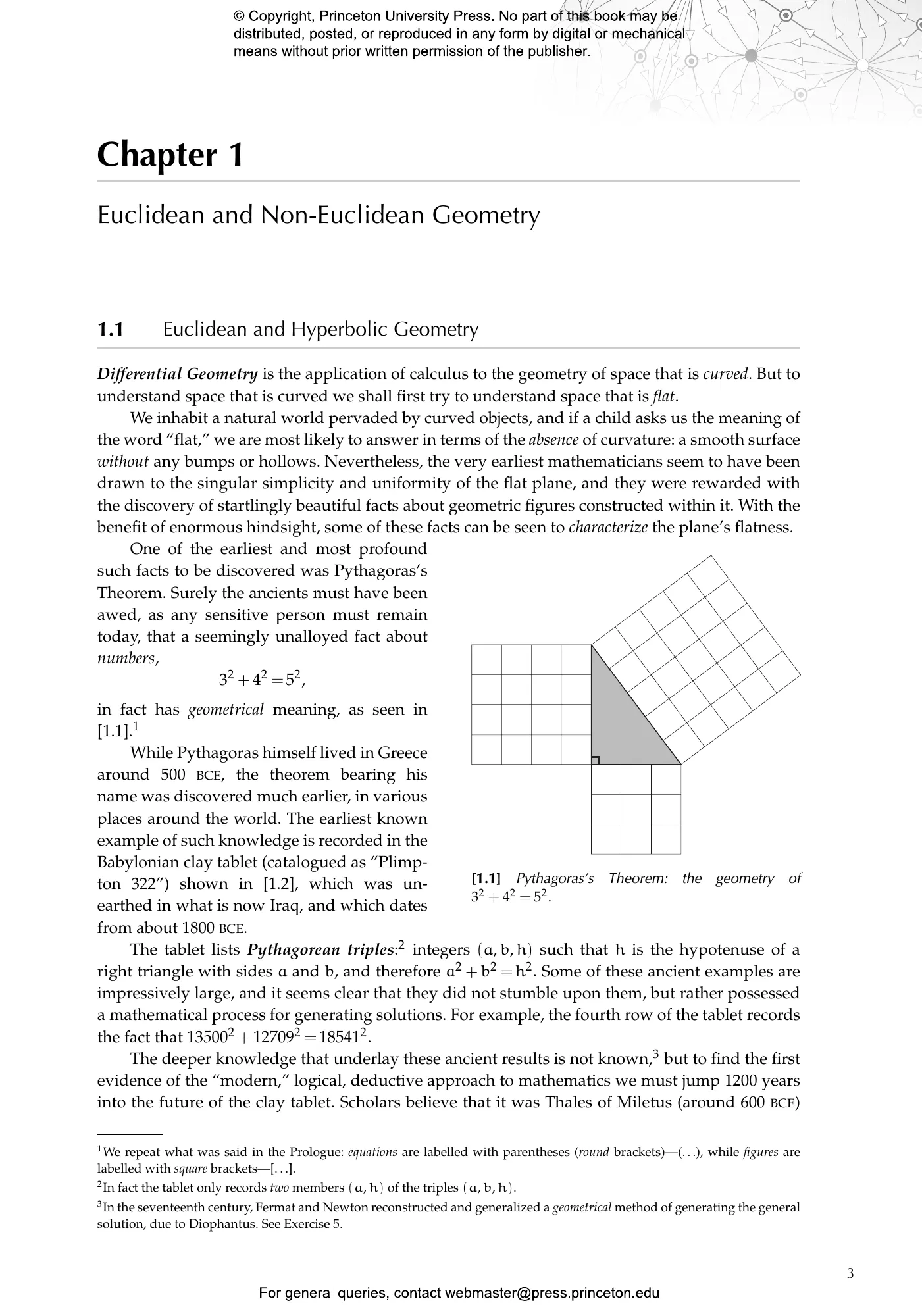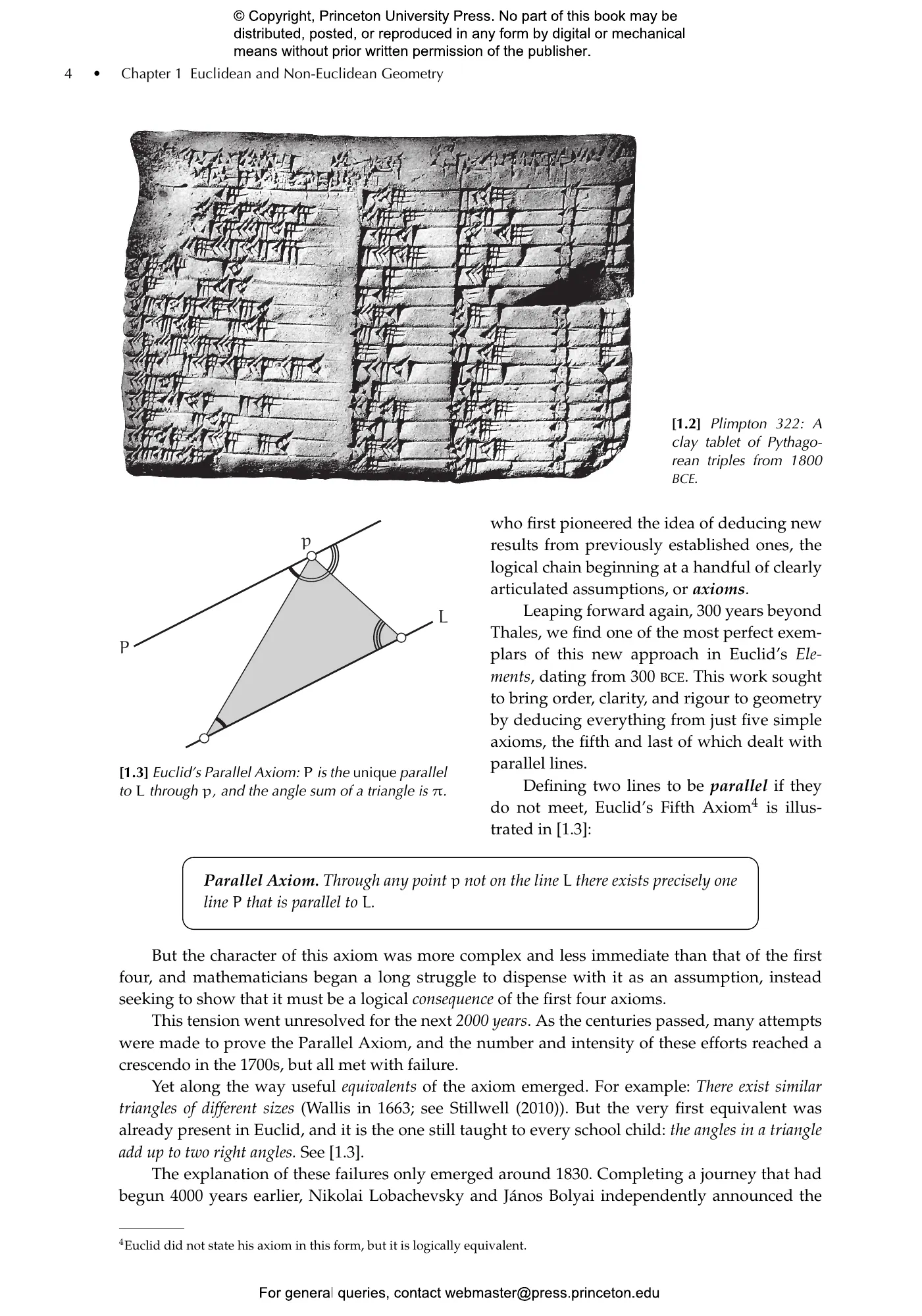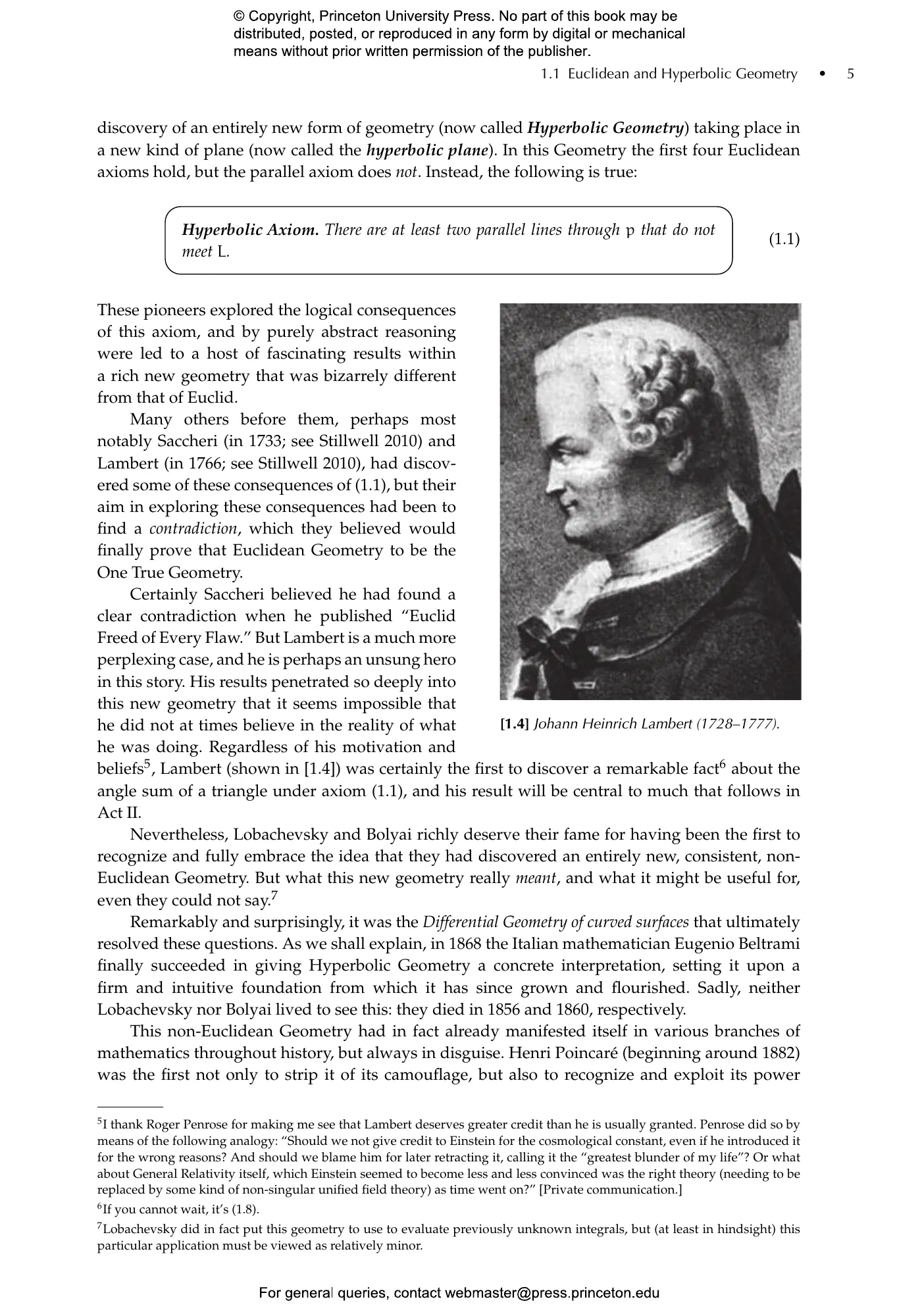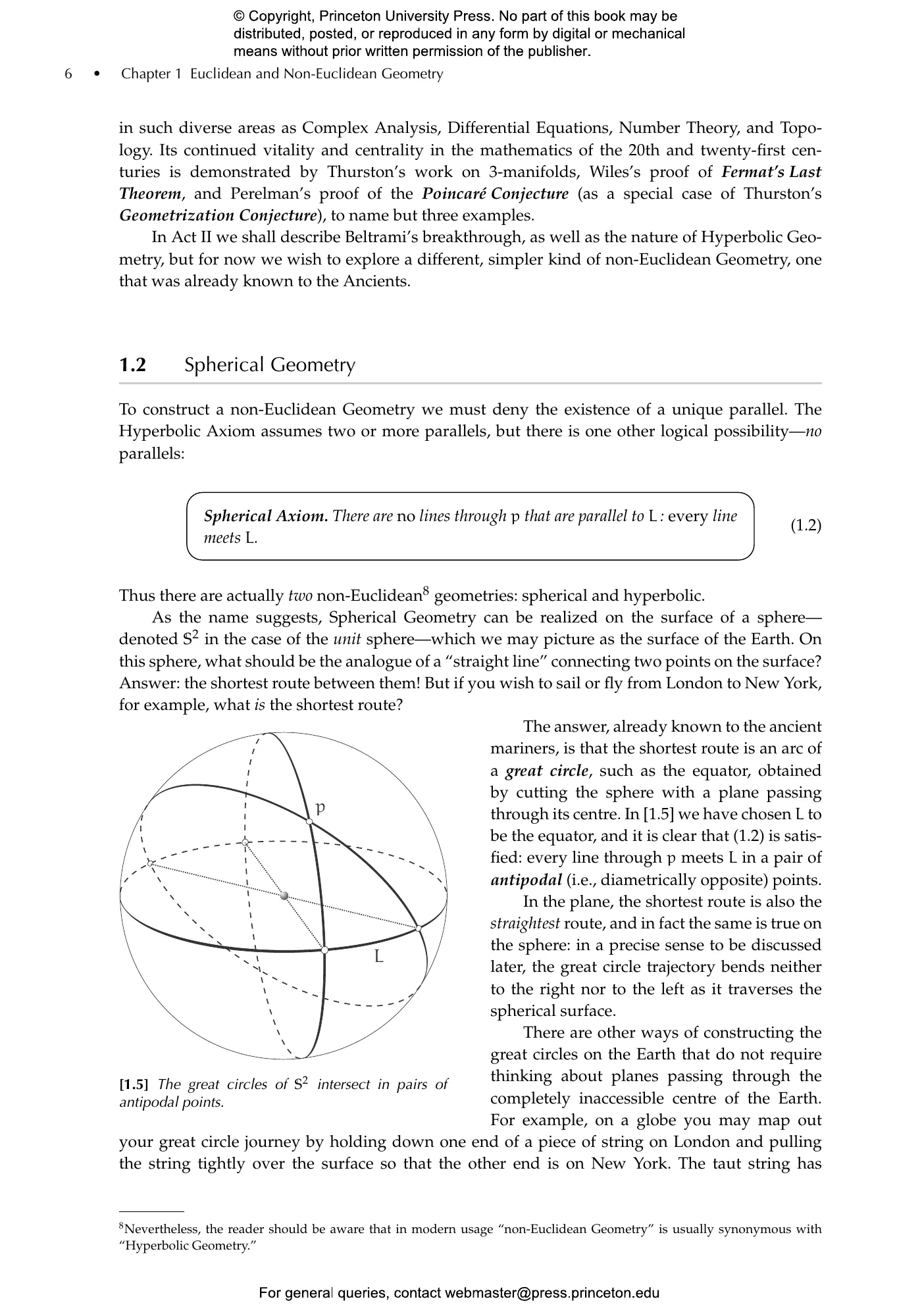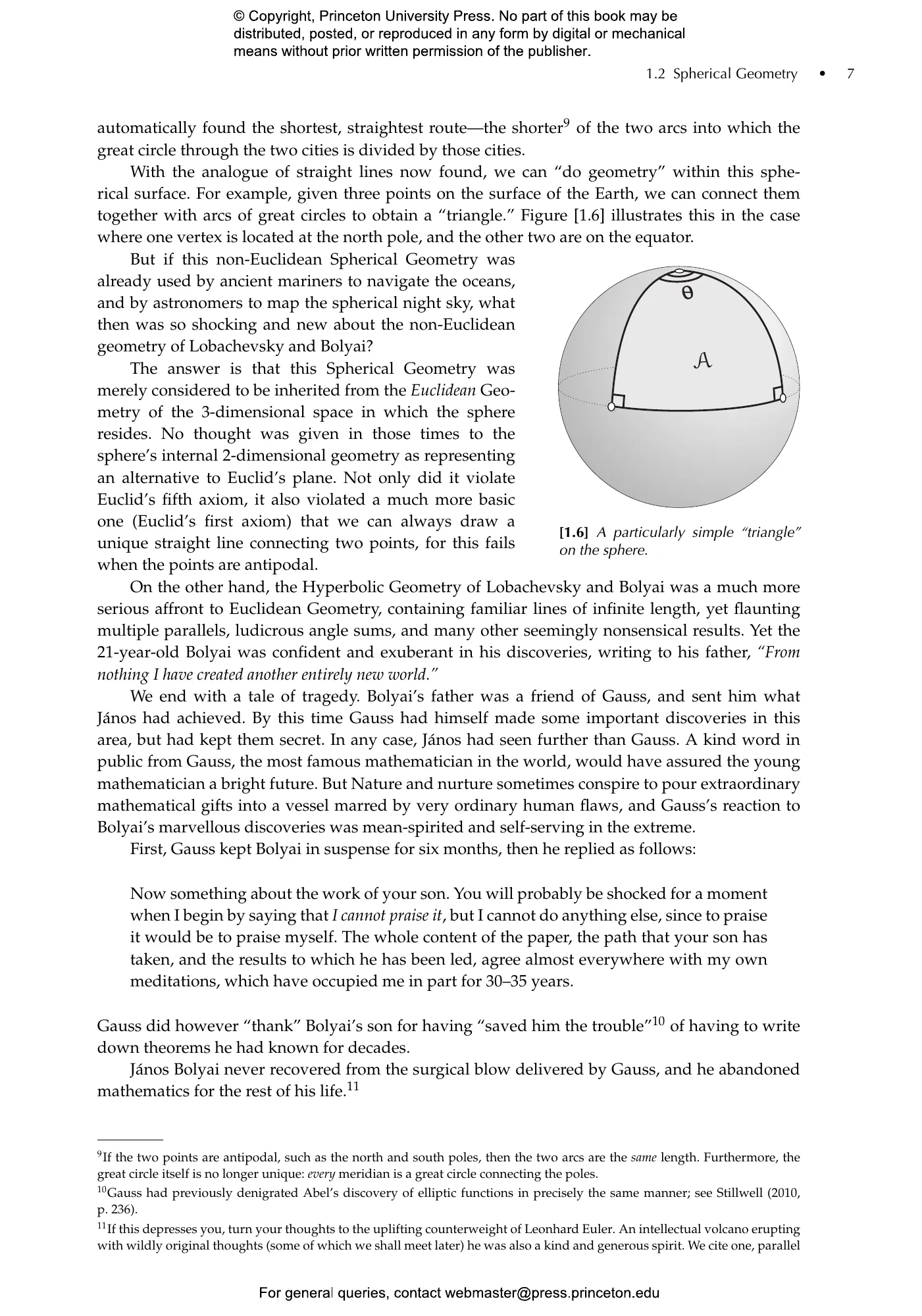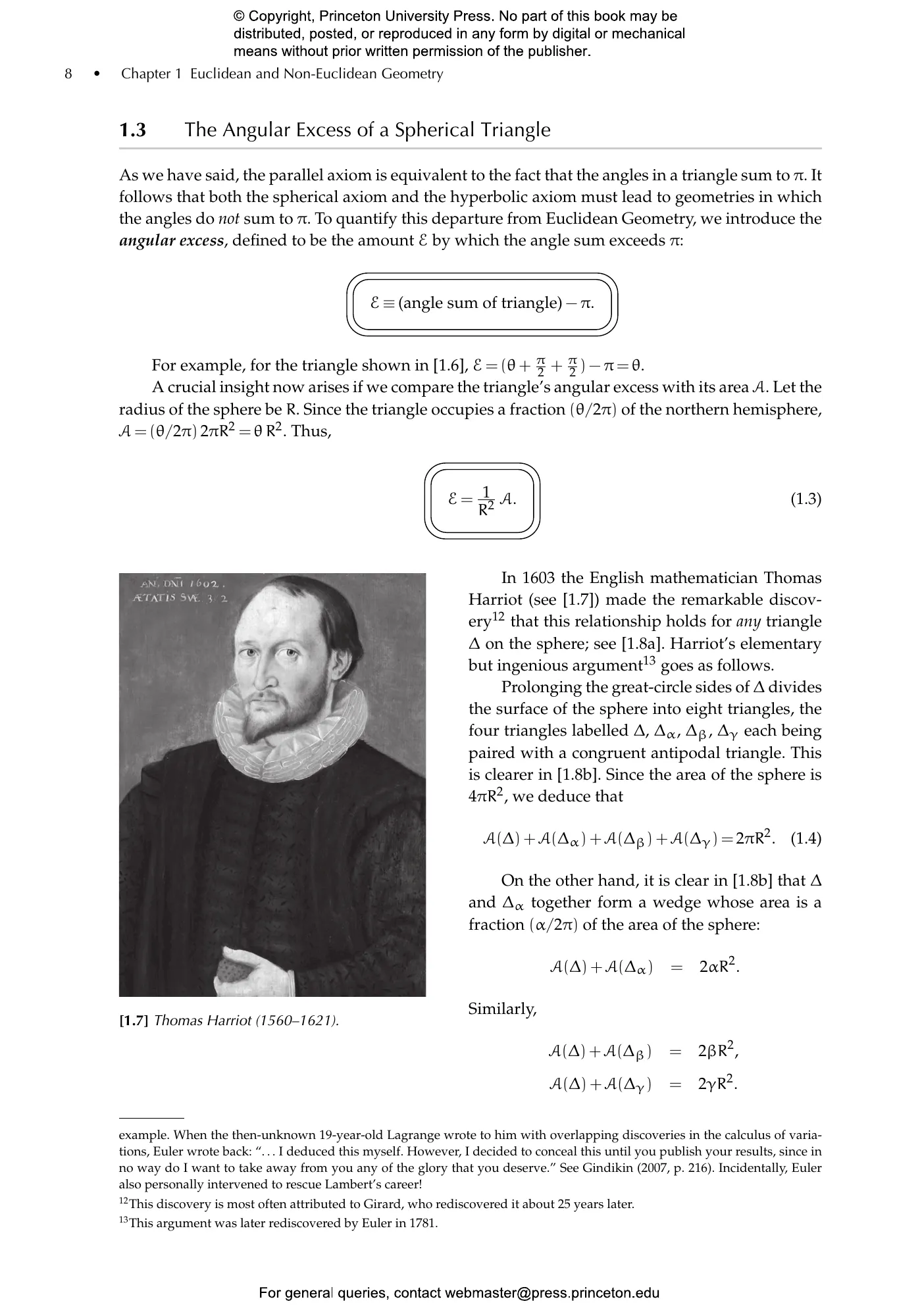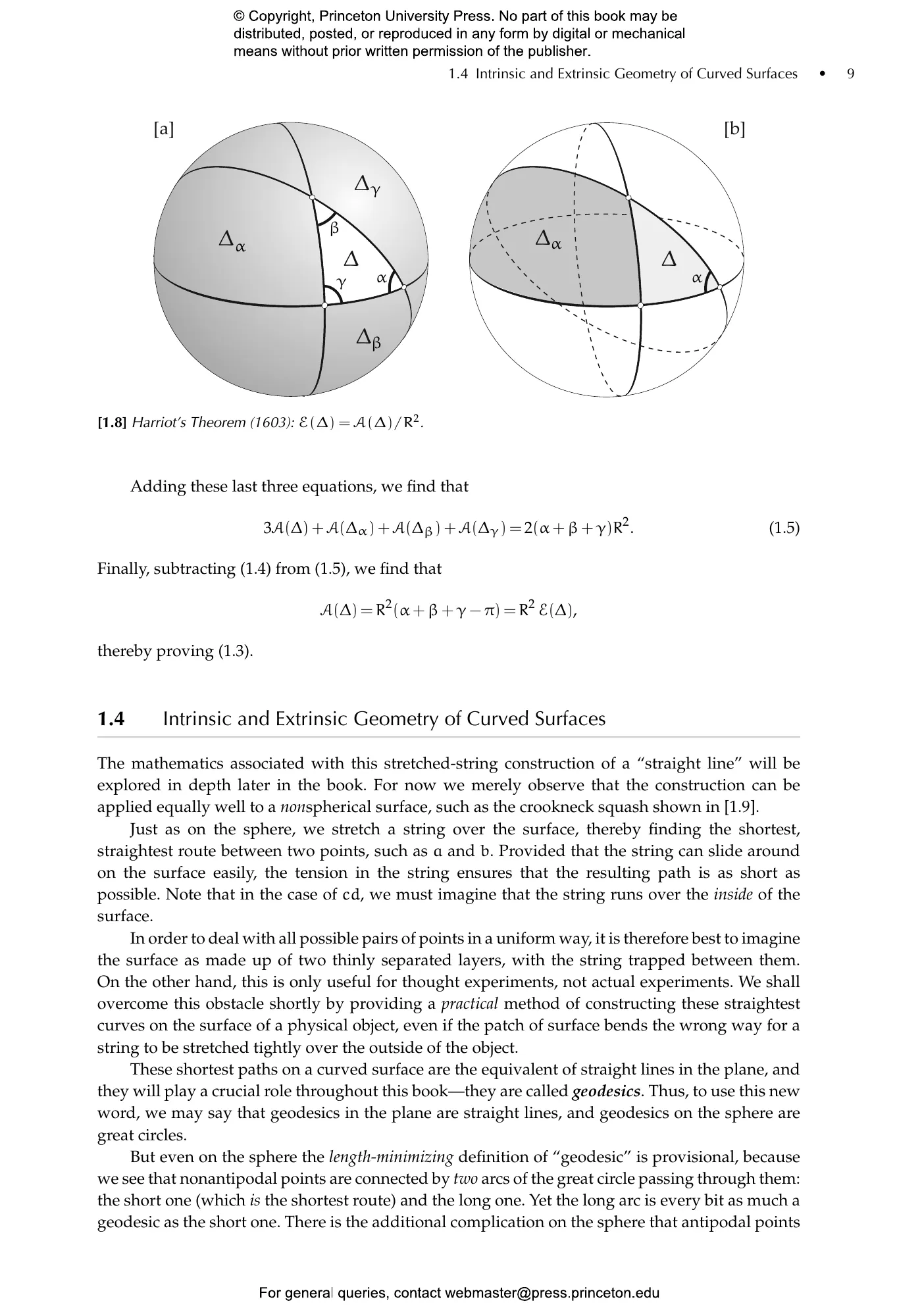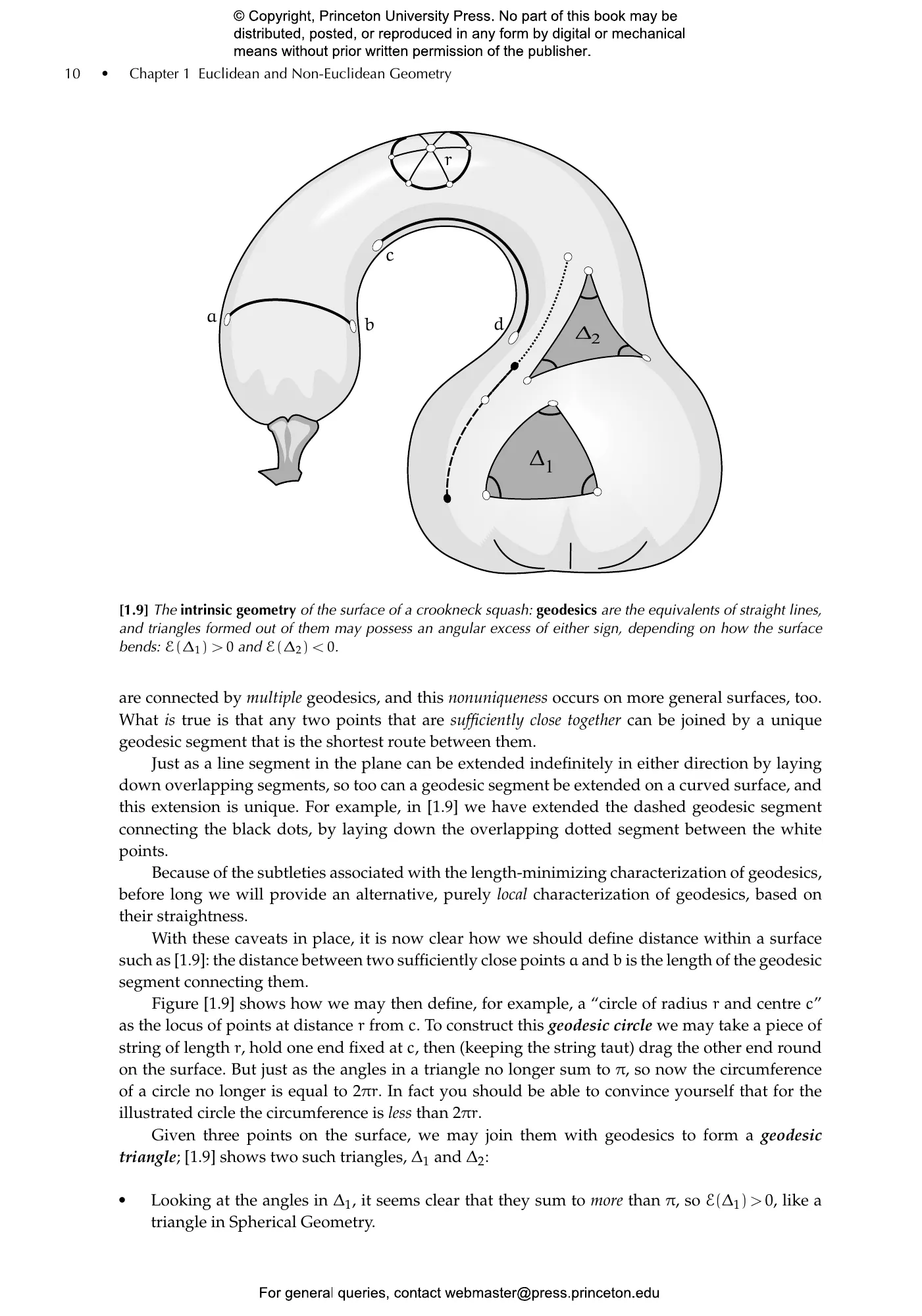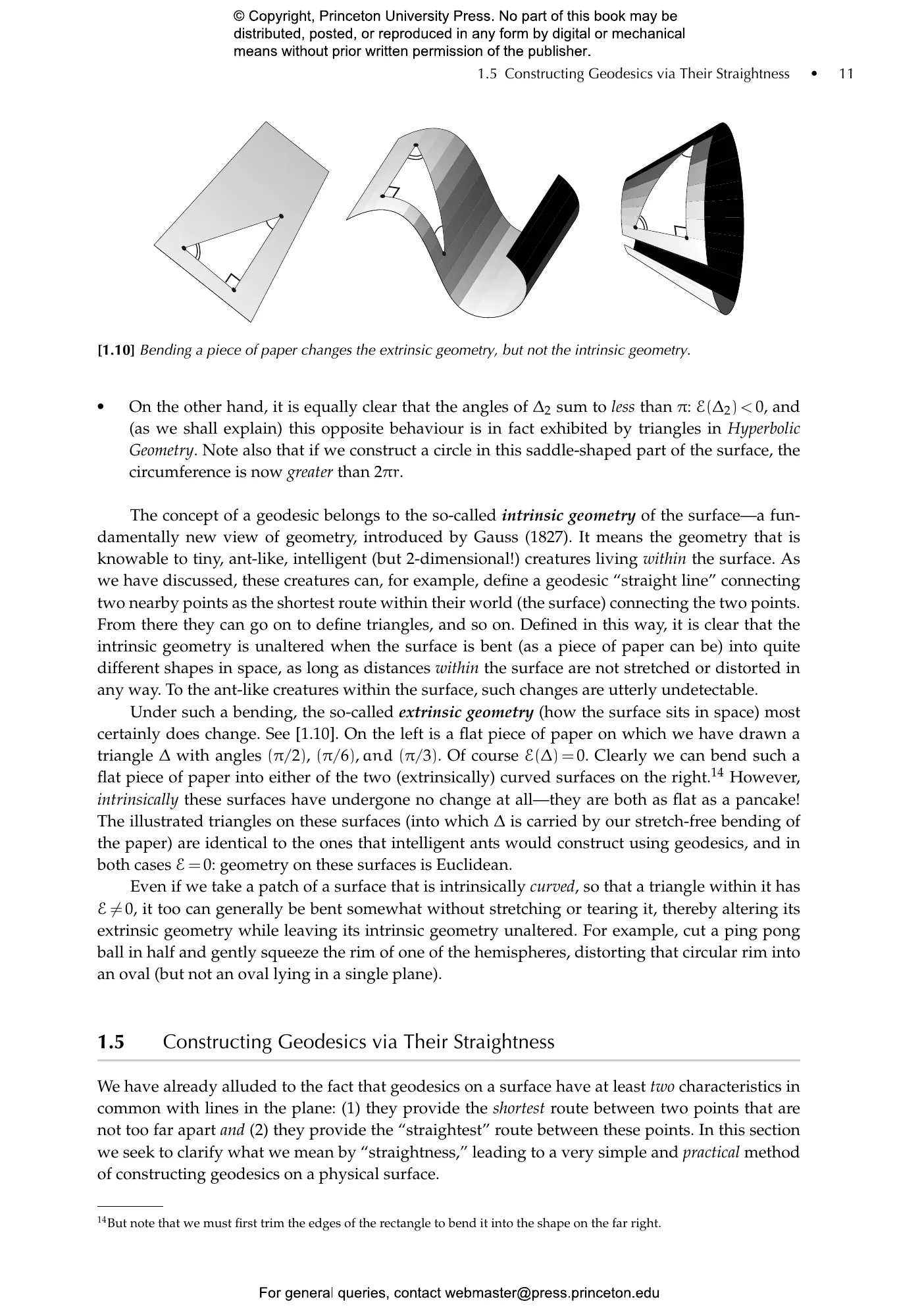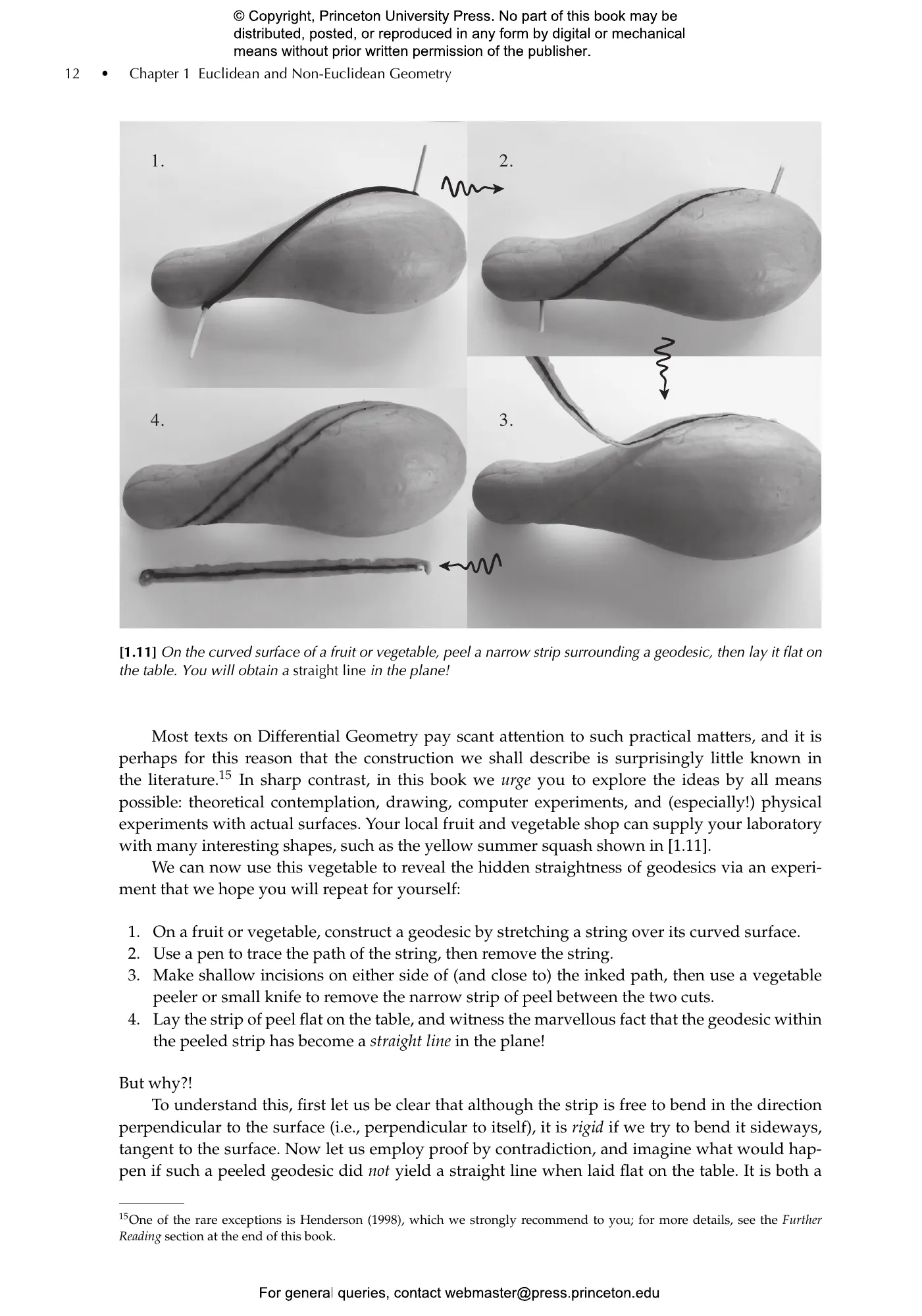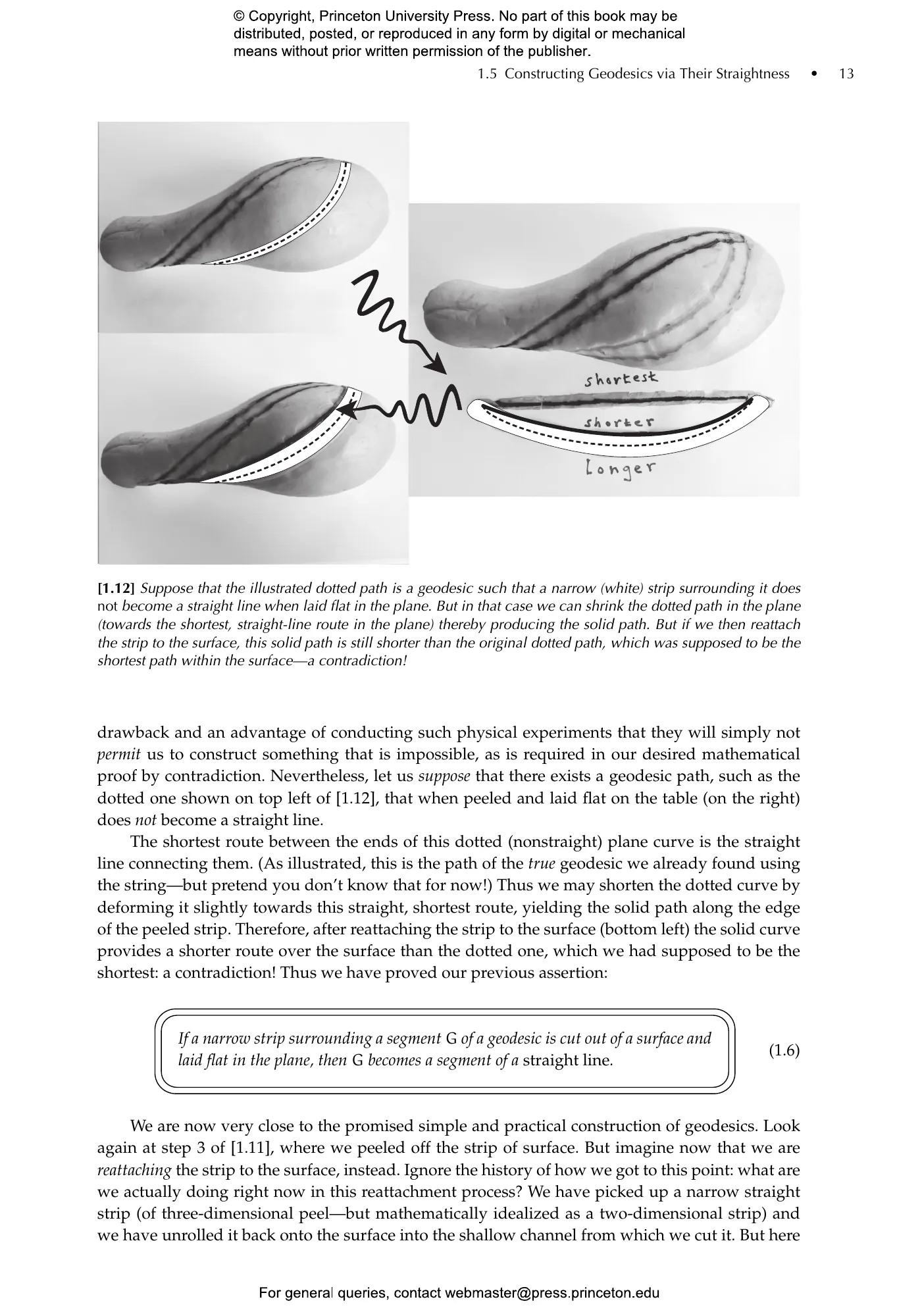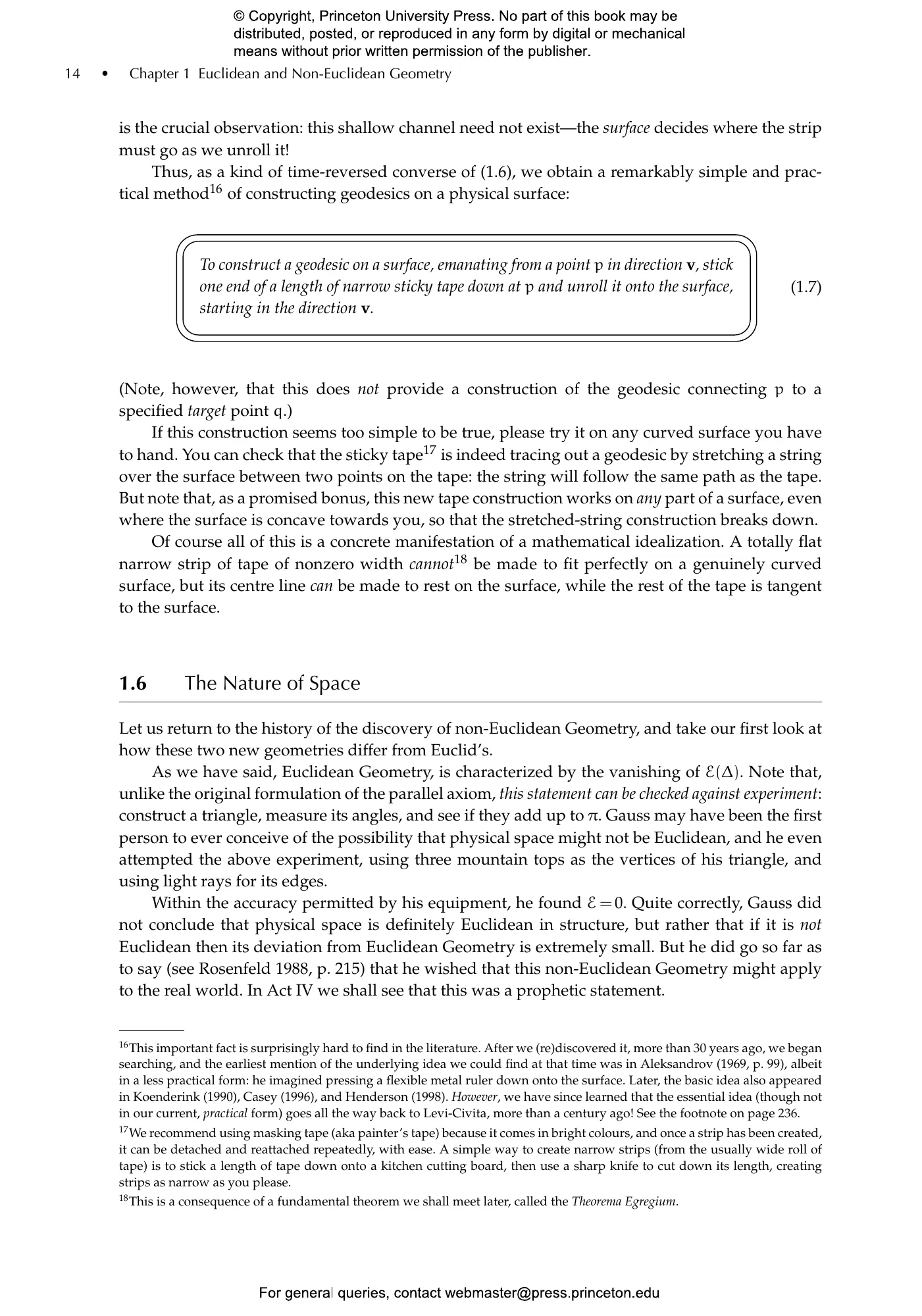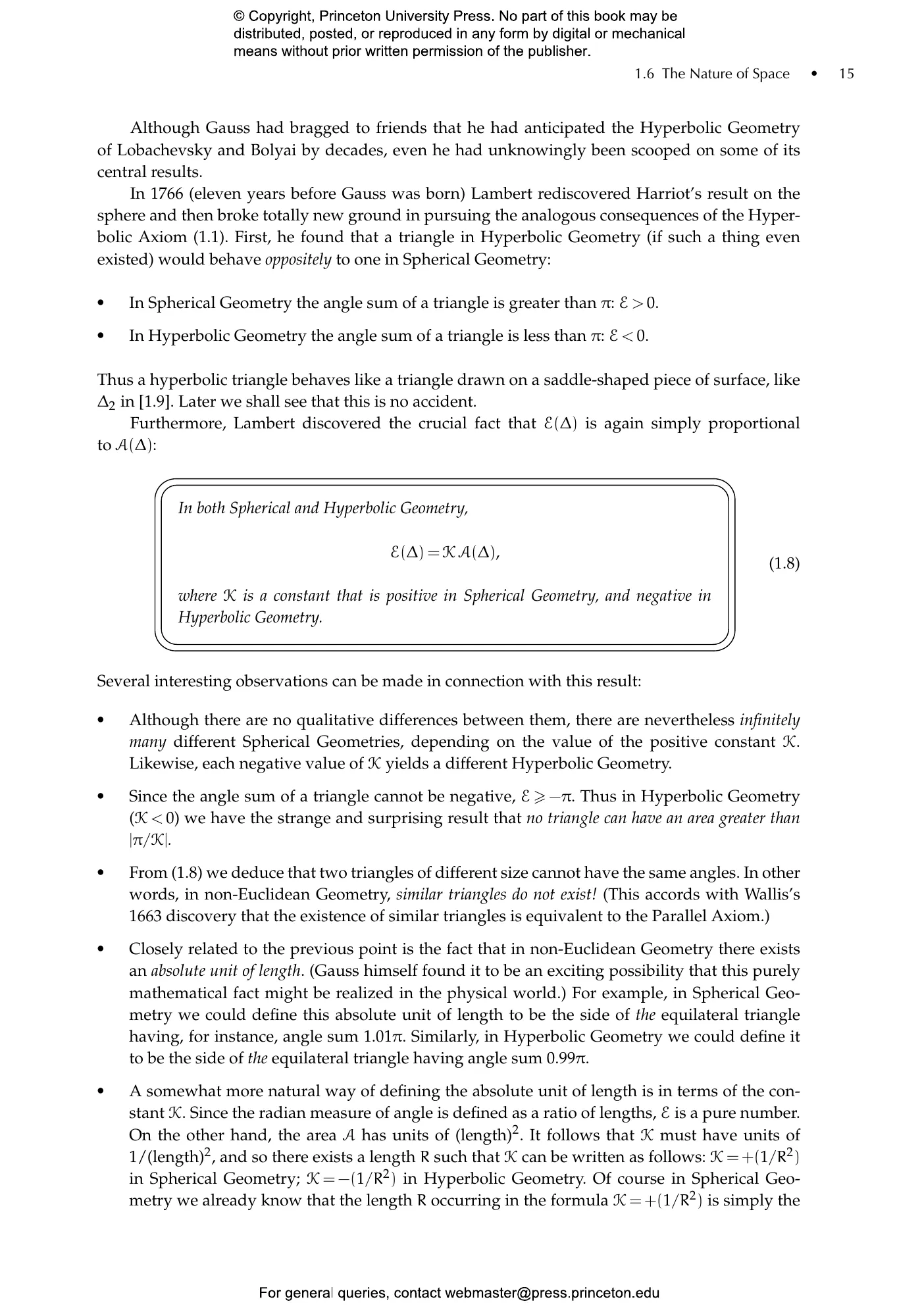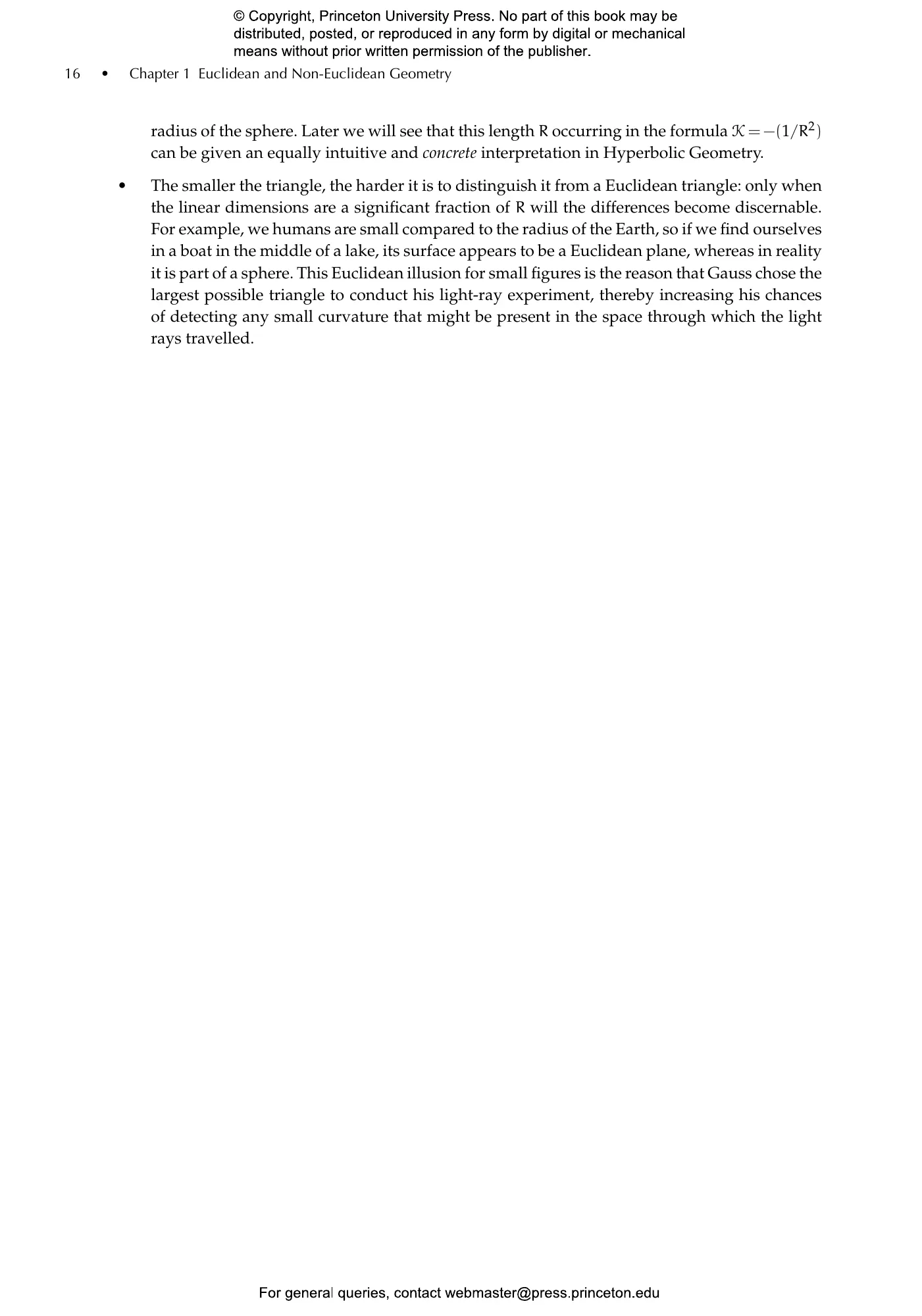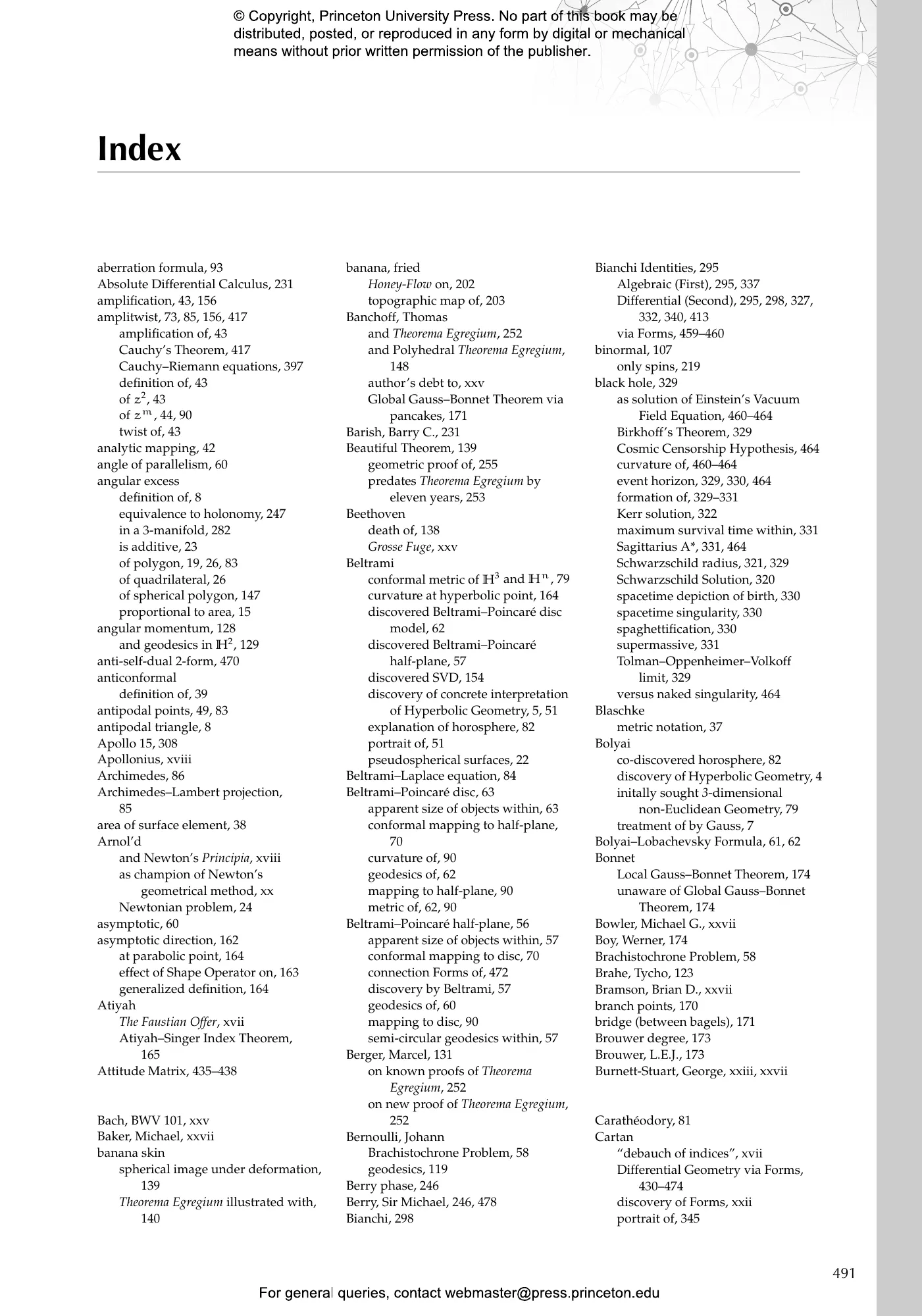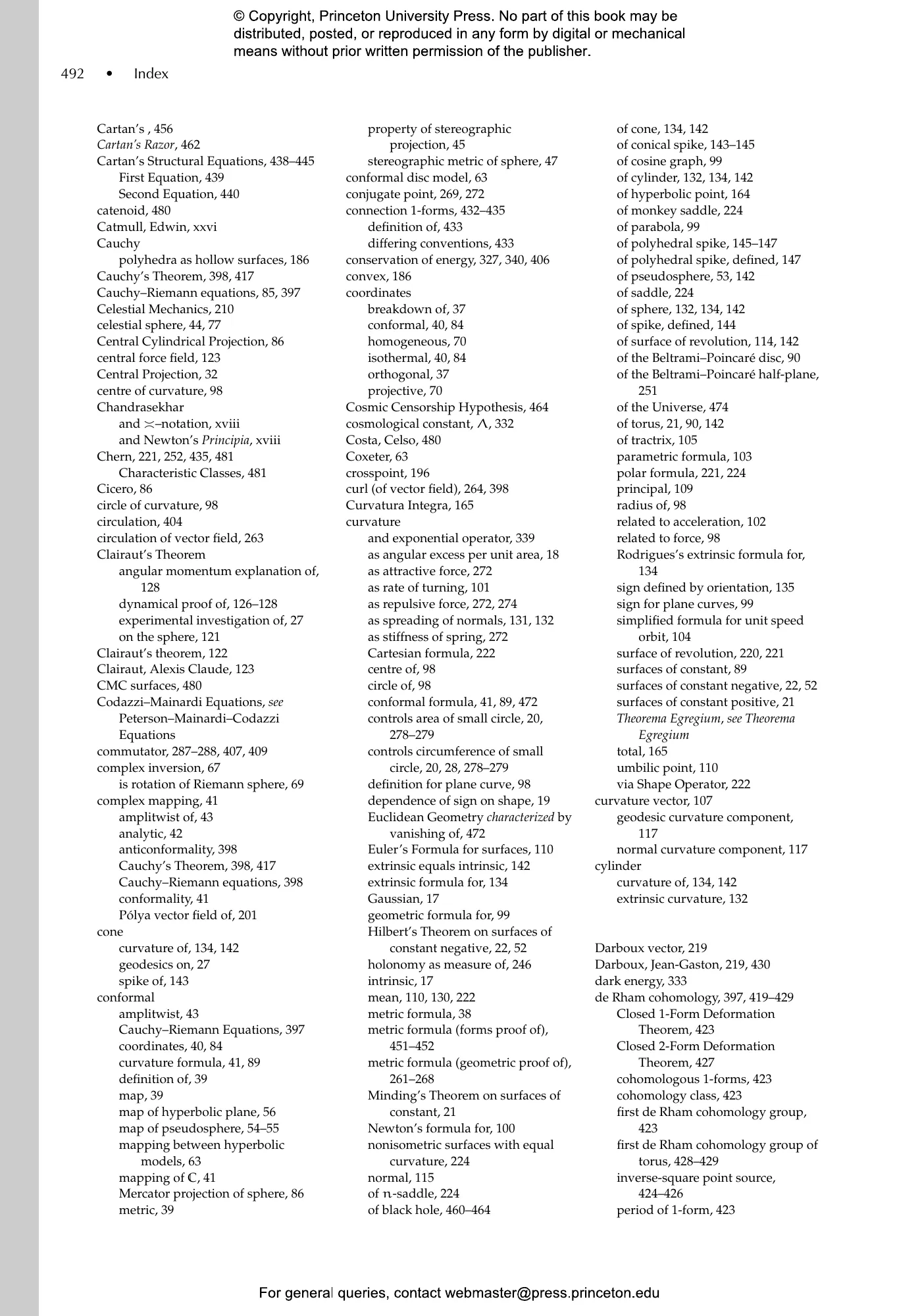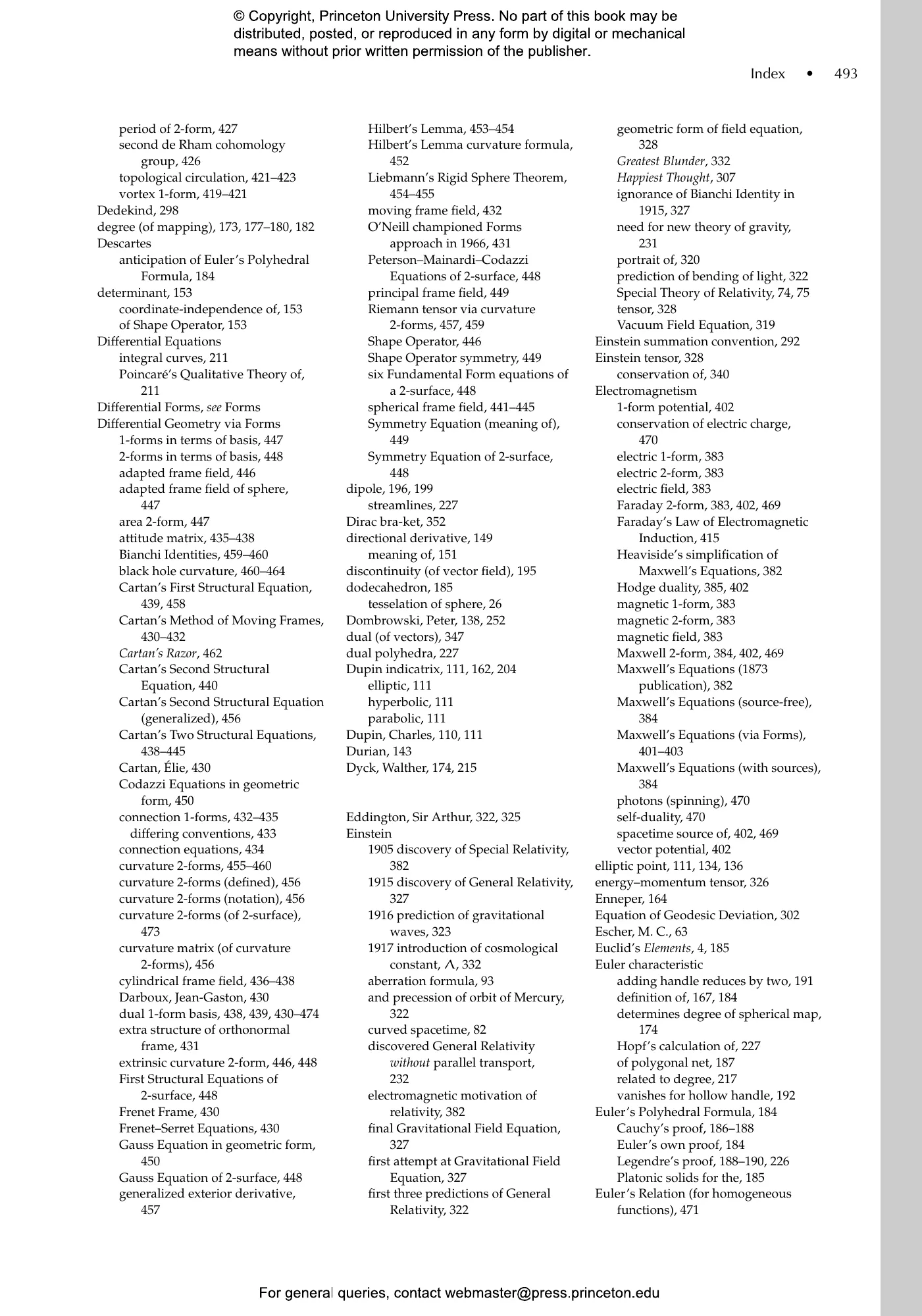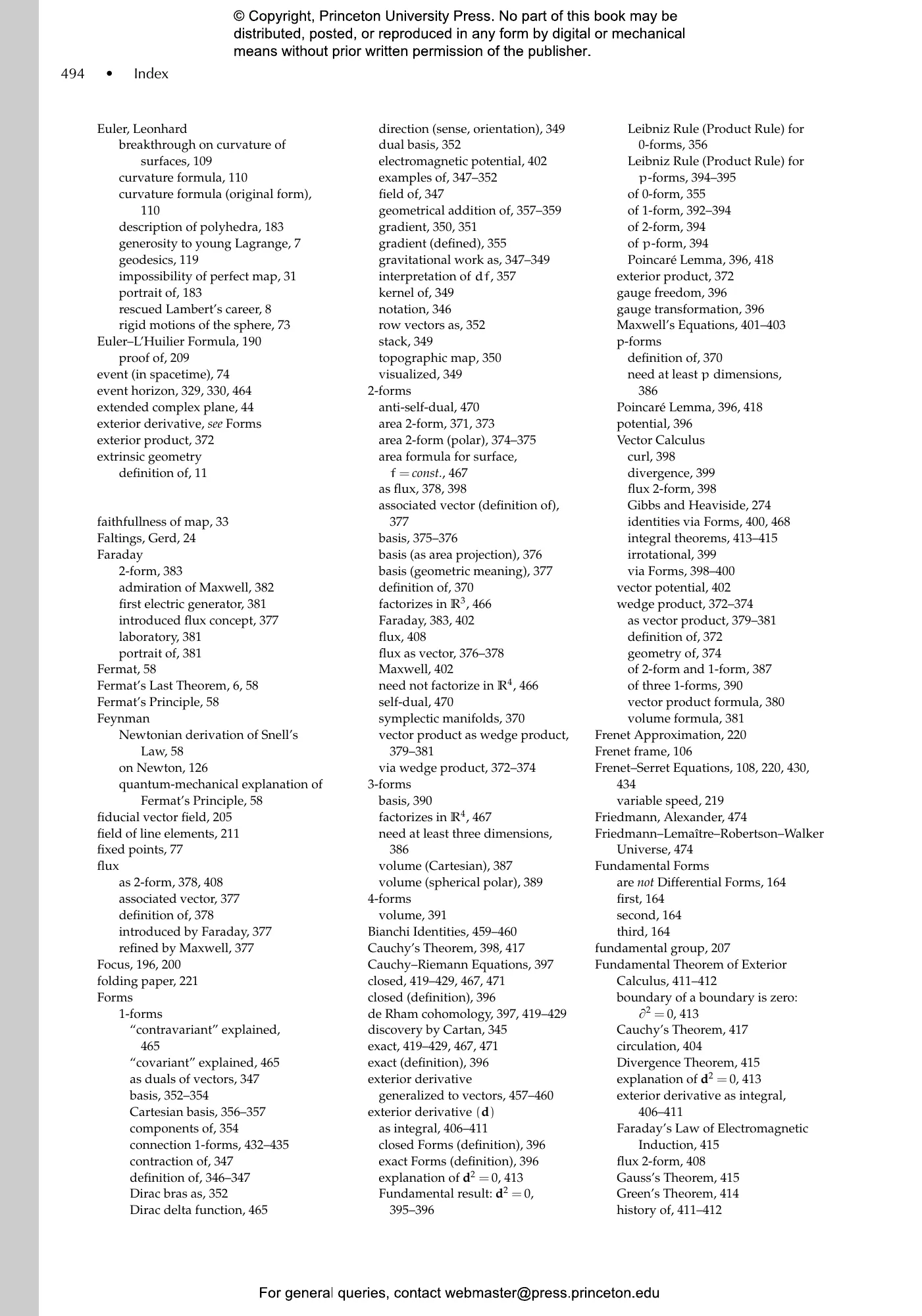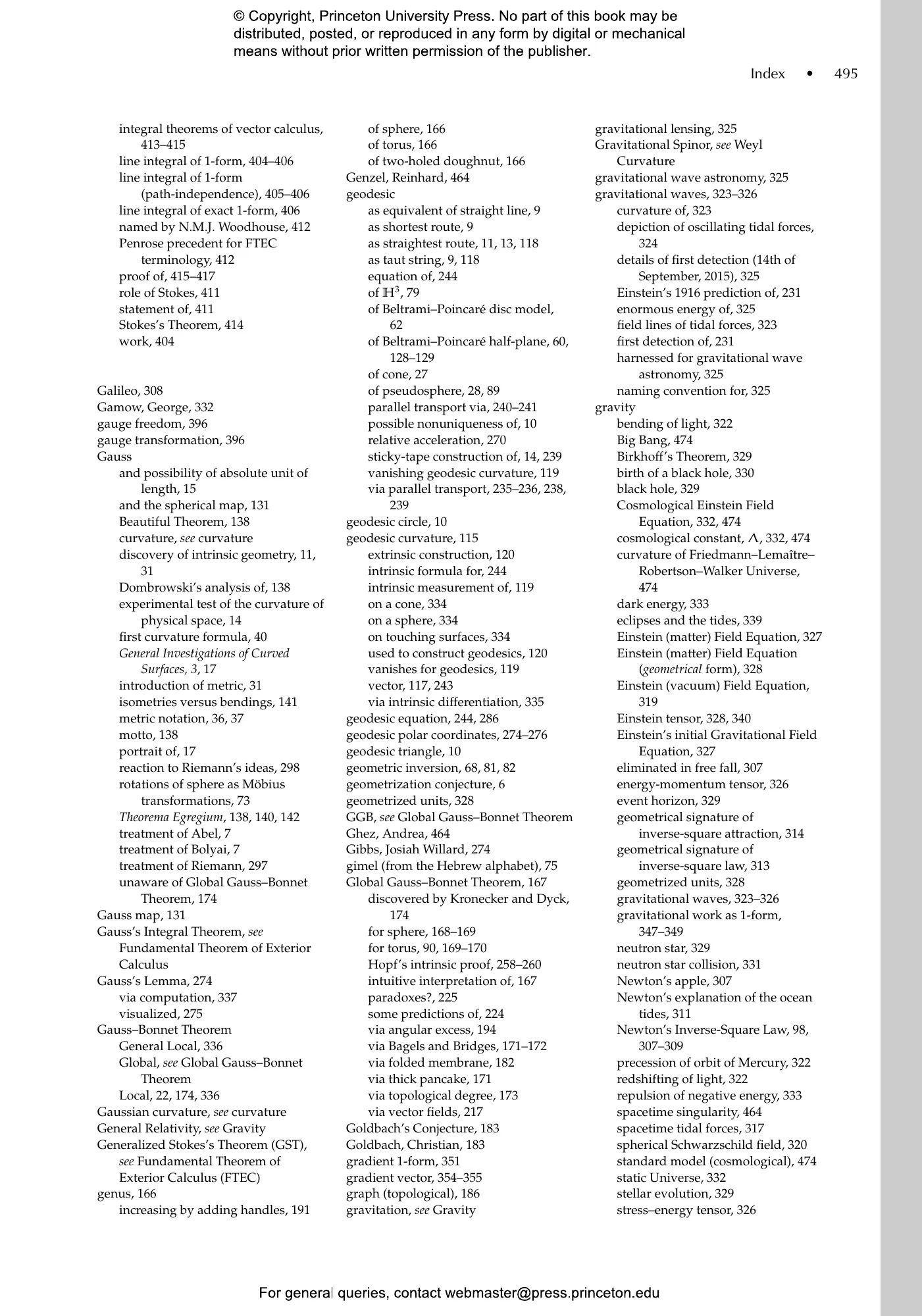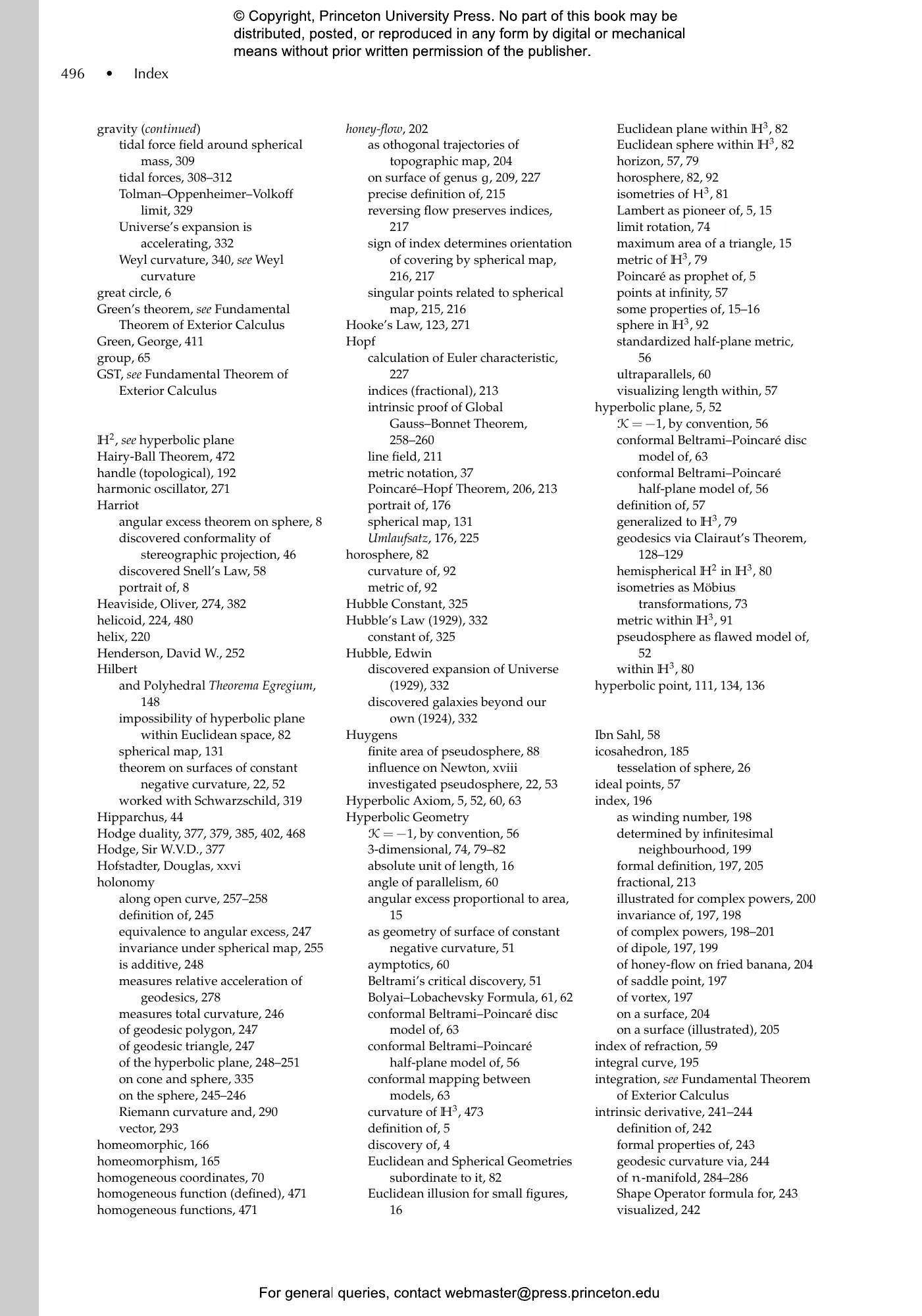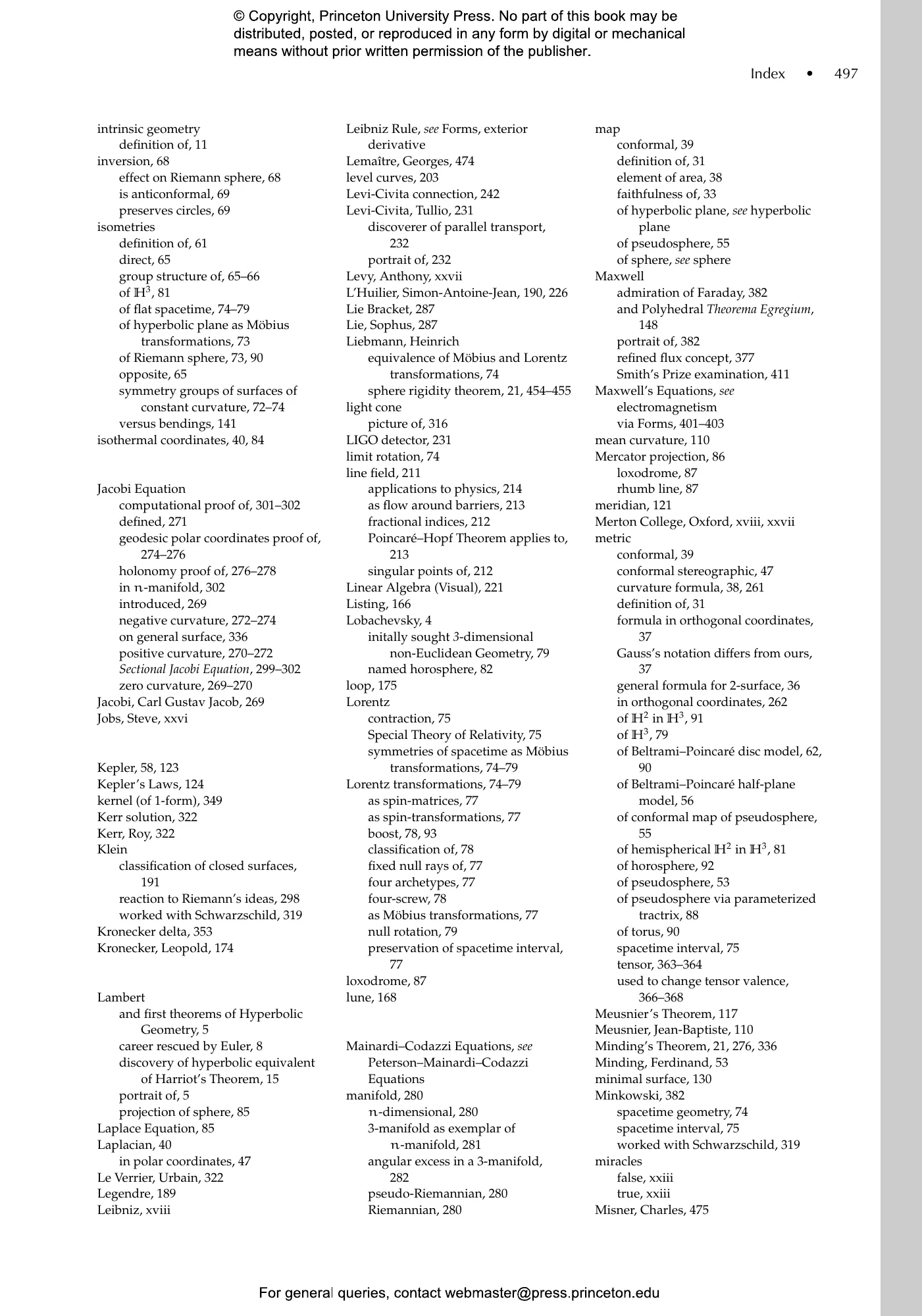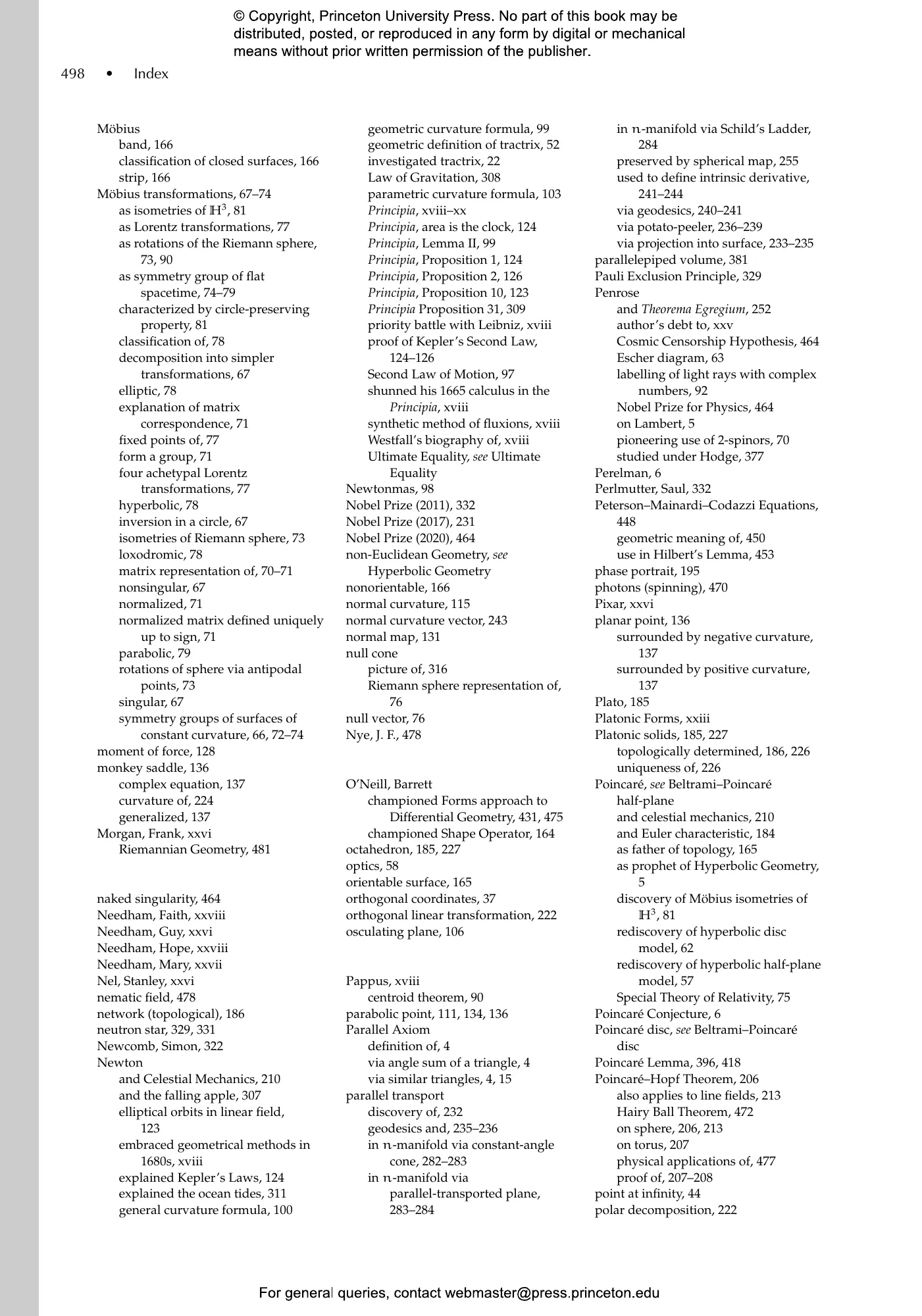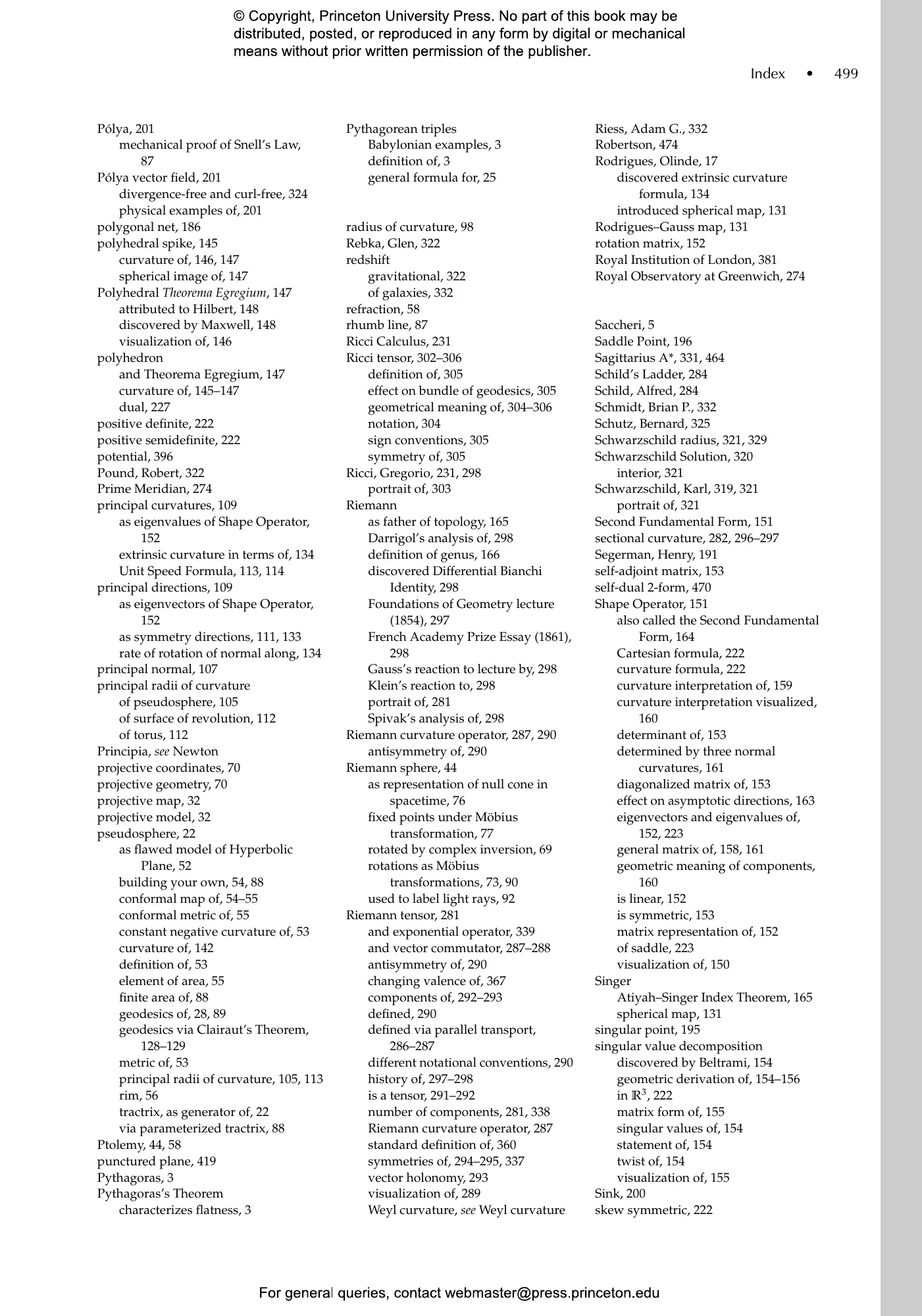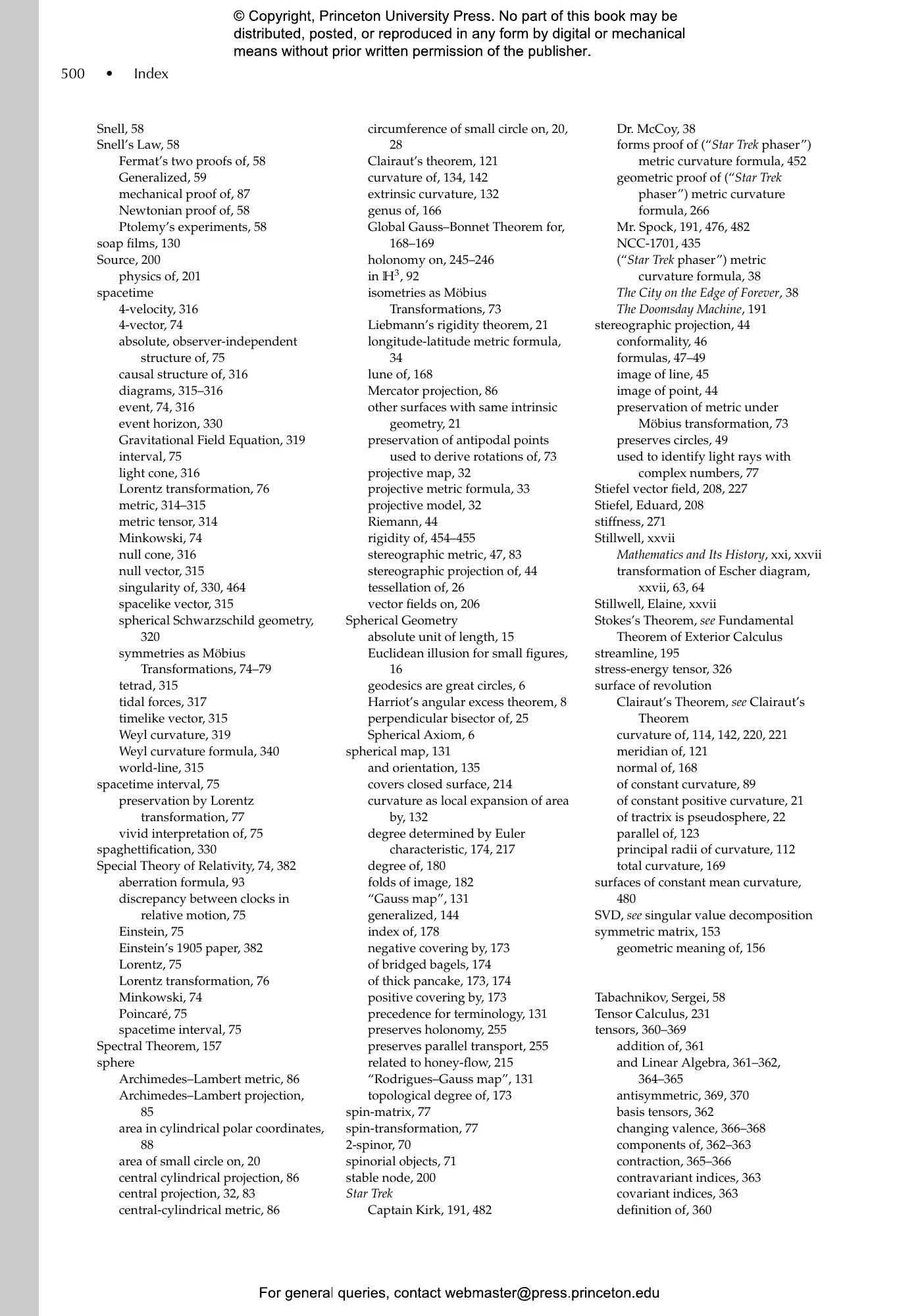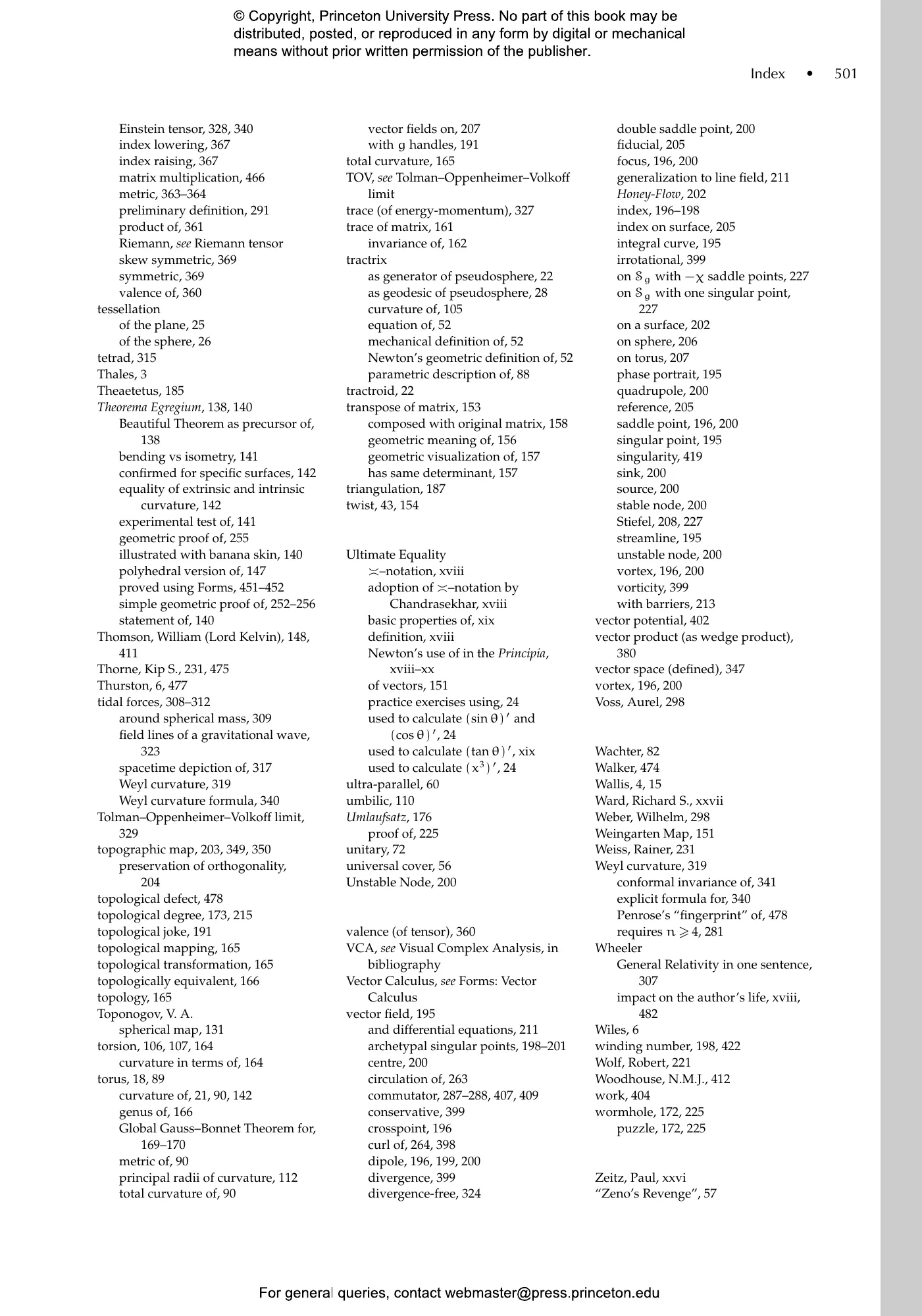#### Visual Differential Geometry and Forms

Tristan Needham

##### ebooks

Many of our ebooks are available for purchase from these online vendors:

Many of our ebooks are available through library electronic resources including these platforms:

# Visual Differential Geometry and Forms: A Mathematical Drama in Five Acts

An inviting, intuitive, and visual exploration of differential geometry and forms## Hardcover

Price:
\$125.00 / £98.00
ISBN:
Published:
Jul 13, 2021
2021
Pages:
584
Size:
7 x 10 in.
Illus:
235 b/w illus.

Visual Differential Geometry and Forms fulfills two principal goals. In the first four acts, Tristan Needham puts the geometry back into differential geometry. Using 235 hand-drawn diagrams, Needham deploys Newton’s geometrical methods to provide geometrical explanations of the classical results. In the fifth act, he offers the first undergraduate introduction to differential forms that treats advanced topics in an intuitive and geometrical manner.

Unique features of the first four acts include: four distinct geometrical proofs of the fundamentally important Global Gauss-Bonnet theorem, providing a stunning link between local geometry and global topology; a simple, geometrical proof of Gauss’s famous Theorema Egregium; a complete geometrical treatment of the Riemann curvature tensor of an n-manifold; and a detailed geometrical treatment of Einstein’s field equation, describing gravity as curved spacetime (General Relativity), together with its implications for gravitational waves, black holes, and cosmology. The final act elucidates such topics as the unification of all the integral theorems of vector calculus; the elegant reformulation of Maxwell’s equations of electromagnetism in terms of 2-forms; de Rham cohomology; differential geometry via Cartan’s method of moving frames; and the calculation of the Riemann tensor using curvature 2-forms. Six of the seven chapters of Act V can be read completely independently from the rest of the book.

Requiring only basic calculus and geometry, Visual Differential Geometry and Forms provocatively rethinks the way this important area of mathematics should be considered and taught.